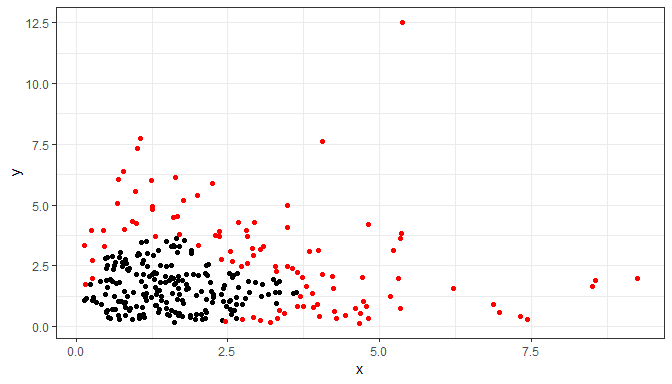User Guide

Preliminaries

We load all the packages used in the examples.

library(ggpmisc)
## Loading required package: ggplot2
## For news about 'ggpmisc', please, see https://www.r4photobiology.info/
library(ggrepel)
library(xts)
## Loading required package: zoo
##
## Attaching package: 'zoo'
## The following objects are masked from 'package:base':
##
##     as.Date, as.Date.numeric
library(lubridate)
##
## Attaching package: 'lubridate'
## The following object is masked from 'package:base':
##
##     date
library(tibble)
library(dplyr)
##
## Attaching package: 'dplyr'
## The following objects are masked from 'package:lubridate':
##
##     intersect, setdiff, union
## The following objects are masked from 'package:xts':
##
##     first, last
## The following objects are masked from 'package:stats':
##
##     filter, lag
## The following objects are masked from 'package:base':
##
##     intersect, setdiff, setequal, union
library(nlme)
##
## Attaching package: 'nlme'
## The following object is masked from 'package:dplyr':
##
##     collapse

As we will use text and labels on the plotting area we change the default theme to an uncluttered one.

old_theme <- theme_set(theme_bw())

Introduction

Many of the functions, including ggplot statistics and geoms, included in package ‘ggpmisc’ had their origin in my need to produce plots for use in teaching or research reports. Some of them are enerally useful, such as stat_poly_eq() and stat_quadrant_counts()but others like stat_fit_deviations() are squarely aimed and producing learning material. Function try_tibble() opens the door to easily converting time series stored in objects of several different classes into data frames. This is, for example, useful for plotting time series data with ggplot(). New ggplot() method specializations for classes ts and xts make the call to try_tibble() and conversion automatic.

ggplot methods for time series

ggplot() methods for classes "ts" and "xts" automate plotting of time series data, as x and y aesthetics are mapped to time and the variable of the time series, respectively. For plotting time series data stored in objects of other classes, see the conversion functions try_tibble() and try_data_frame() in the last section of this vignette.

class(lynx)
##  "ts"
ggplot(lynx) + geom_line()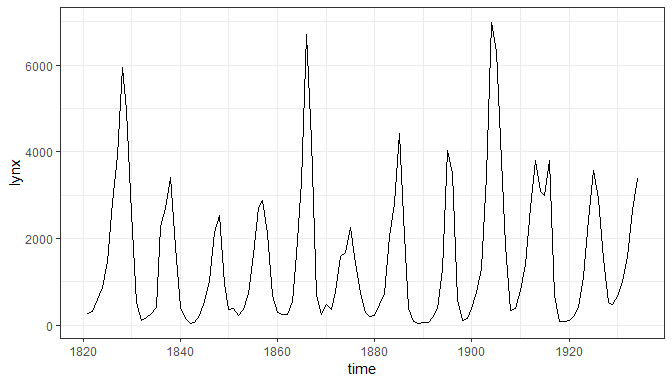It is possible to control the conversion of the time variable to numeric or datetime. As we will see below this affects the scale used by default as well as the formatting of values when converted to character strings or printed.

ggplot(lynx, as.numeric = FALSE) + geom_line()geom_table and stat_fmt_table

The geometry geom_table() plots a data frame or tibble, nested in a tibble passed as data argument, using aesthetics x and y for positioning, and label for the data frame containing the data for the table. The table is created as a ‘grid’ grob and added as usual to the ggplot object. In contrast to “standard” geoms, this geom by default does not inherit the globally mapped aesthetics.

tb <- mpg %>%
group_by(cyl) %>%
summarise(hwy = median(hwy), cty = median(cty))
data.tb <- tibble(x = 7, y = 44, tb = list(tb))
ggplot(mpg, aes(displ, hwy, colour = factor(cyl))) +
geom_table(data = data.tb, aes(x, y, label = tb)) +
geom_point()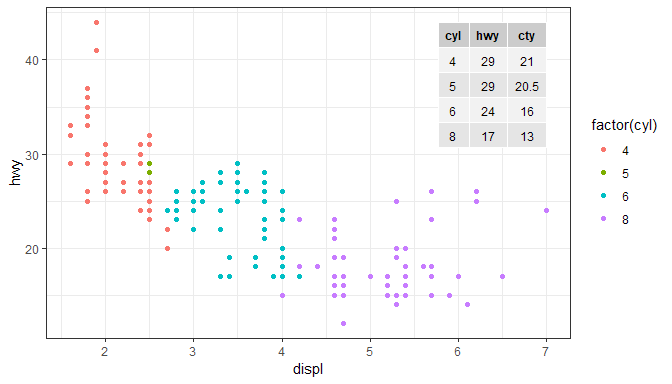Using stat_fmt_tb() we can rename columns of the tibble, and/or select a subset of columns or a slice of rows as shown below. To provide a complete example we also replace the names of the x, y and colour aesthetics.

ggplot(mpg, aes(displ, hwy, colour = factor(cyl))) +
geom_table(data = data.tb, aes(x, y, label = tb),
size = 3, colour = "dark blue",
stat = "fmt_tb", tb.vars = c(Cylinders = "cyl", "MPG" = "hwy")) +
labs(x = "Engine displacement (l)", y = "Fuel use efficiency (MPG)",
colour = "Engine cylinders\n(number)") +
geom_point() +
theme_bw()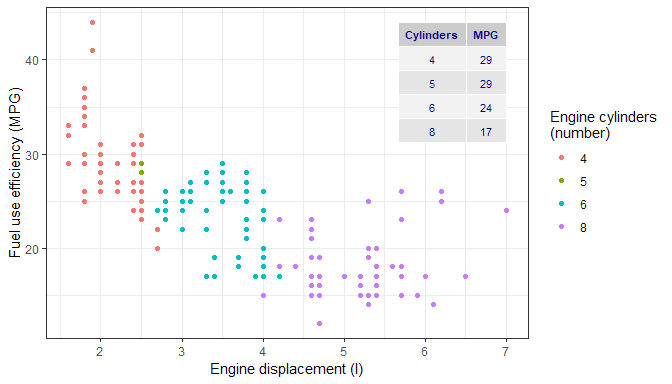Parsed text, using plot math syntax is supported in the table, with fall-back to plain text in case of parsing errors, on a cell by cell basis. Here we plot the MPG for city traffic and we can see that the plotting area expands to include the coordinates at which the table is anchored. Justification is by default set to "inward" which ensures that the table is fully within the plotting region.

tb.pm <- tibble(Parameter = c("frac(beta, a^2)", "frac(beta, a^3)"),
Value = c("10^2.4", "10^3.532"))
data.tb <- tibble(x = 7, y = 44, tb = list(tb.pm))
ggplot(mpg, aes(displ, cty)) +
geom_point() +
geom_table(data = data.tb, aes(x, y, label = tb), parse = TRUE) +
theme_bw()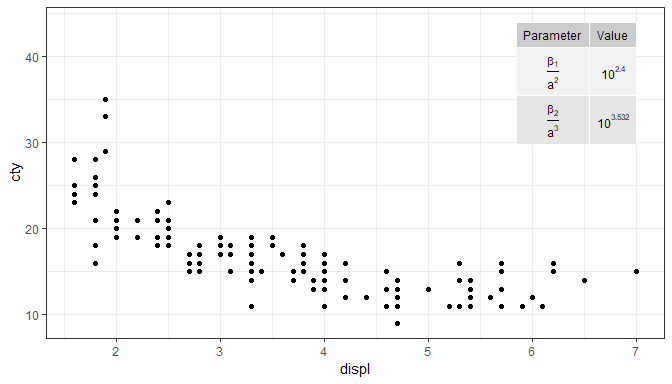As implemented, there is no limitation to the number of insets, and faceting is respected. If the base plot shows a map, multiple small tables could be superimposed on different countries or regions. The size of the insets is set relative to the main plot, so the combined plot can be scaled.

Please see section Normalised Parent Coordinates below for a description of geom_table_npc().

geom_plot

The geom_plot() geometry plots ggplot objects, nested in a tibble passed as data argument, using easthetics x and y for positioning, and label for the ggplot object containing the definition of the plot to be nested. A Grob is created with ‘ggplotGrob’ and added as usual to the ggplot object.

As an example we produce plot where the inset plot is a zommed-in detail from the main plot. In this case the main and inset plots start as the same plot.

p <- ggplot(mpg, aes(displ, hwy, colour = factor(cyl))) +
geom_point()

data.tb <- tibble(x = 7, y = 44,
plot = list(p +
coord_cartesian(xlim = c(4.9, 6.2), ylim = c(13, 21)) +
labs(x = NULL, y = NULL) +
theme_bw(8) +
scale_colour_discrete(guide = FALSE)))

ggplot(mpg, aes(displ, hwy, colour = factor(cyl))) +
geom_plot(data = data.tb, aes(x, y, label = plot)) +
annotate(geom = "rect",
xmin = 4.9, xmax = 6.2, ymin = 13, ymax = 21,
linetype = "dotted", fill = NA, colour = "black") +
geom_point()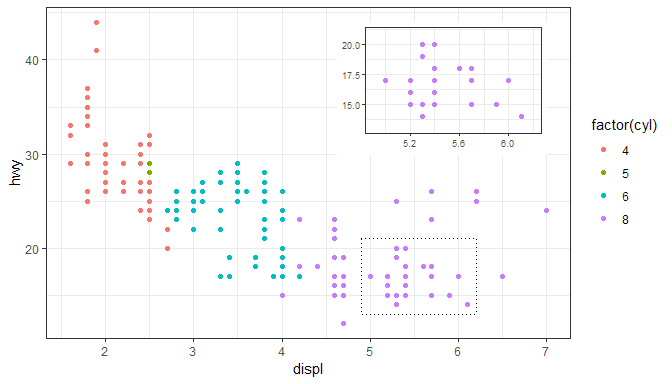In general, the inset plot can be any ggplot object, allowing the creation of very different combinations of main plot and inset plot. Here we use the inset to summaries as in the previous example of an inset table.

p <- ggplot(mpg, aes(factor(cyl), hwy, fill = factor(cyl))) +
stat_summary(geom = "col", fun.y = mean, width = 2/3) +
labs(x = "Number of cylinders", y = NULL, title = "Means") +
scale_fill_discrete(guide = FALSE)

data.tb <- tibble(x = 7, y = 44,
plot = list(p +
theme_bw(8)))

ggplot(mpg, aes(displ, hwy, colour = factor(cyl))) +
geom_plot(data = data.tb, aes(x, y, label = plot)) +
geom_point() +
labs(x = "Engine displacement (l)", y = "Fuel use efficiency (MPG)",
colour = "Engine cylinders\n(number)") +
theme_bw()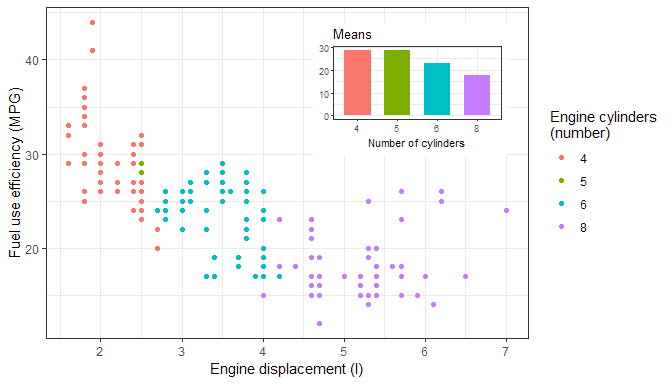As implemented, there is no limitation to the number of insets, and faceting is respected. If the base plot shows a map, multiple small plots could be superimposed on different countries or regions. The size of the insets is set relative to the main plot, so the combined plot can be scaled. A possible unintuitive but useful feature, is that the theme is linked to each plot.

Please see section Normalised Parent Coordinates below for a description of geom_plot_npc().

geom_grob

The geom_grob() geometry plots grobs (graphical objects as created with ‘grid’), nested in a tibble passed as data argument, using easthetics x and y for positioning, and label for the Grob object. While geom_table() and geom_plot() take as values to plot tibbles or data frames, and ggplots, respectively, and convert them into Grobs before adding them to the plot, geom_grob() expects Grobs ready to be rendered.

file.name <-
system.file("extdata", "Isoquercitin.png",
package = "ggpmisc", mustWork = TRUE)
grobs.tb <- tibble(x = c(0, 10, 20, 40), y = c(4, 5, 6, 9),
width = c(0.05, 0.05, 0.01, 1),
height =  c(0.05, 0.05, 0.01, 0.3),
grob = list(grid::circleGrob(),
grid::rectGrob(),
grid::textGrob("I am a Grob"),
grid::rasterGrob(image = Isoquercitin)))

ggplot() +
geom_grob(data = grobs.tb,
aes(x, y, label = grob, vp.width = width, vp.height = height),
hjust = 0.7, vjust = 0.55) +
scale_y_continuous(expand = expand_scale(mult = 0.3, add = 0)) +
scale_x_continuous(expand = expand_scale(mult = 0.2, add = 0)) +
theme_bw(12)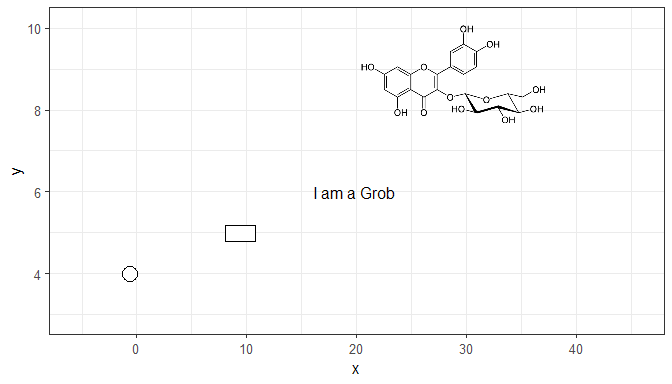geom_grob() is designed thinking that its main use will in graphical annotations, although one could use it for infographics with multiple copies of each grob, this would go against the grammar of graphics. In this implementation grobs cannot to mapped to an aesthetic through a scale.

As implemented, there is no limitation to the number of insets, and faceting is respected. If the base plot shows a map, multiple simple grobs (e.g. national flags) could be superimposed on different countries. The size of the insets is set relative to the main plot, so the combined plot can be scaled.

Please see next section for a description of geom_grob_npc().

Normalised Parent Coordinates

R’s ‘grid’ pakage defines several units that can be used to describe the locations of plot elements. In ‘ggplot2’ the x and y aesthetics are directly mapped to "native" or data units. For consistent location of annotations with respect to the plotting area we need to rely on "npc" which are expressed relative to the size of the grid viewport, as the plotting area in a ggplot if implemented as viewport.

To support this we have implemented scales for two new aesthetics, npcx and npcy. These are very simple continuous scales which do not support any transformation or change to their limits, which are meaningless for "npc" units. Variables mapped to these aesthetics can be either numerical with values in the range zero to one or character. A limited set of strings are recognised and converted to “npc” units: "top", "bottom", "left", "right" and "centre" (or its synonims "center" and "middle"). Justification defaults to “inward”.

To make these scales useful we need also to define geometries that use these new aesthetics. Package ‘ggpmisc’ currently provides geom_text_npc(), geom_label_npc(), geom_table_npc(), geom_plot_npc() and geom_grob_npc().

These are used internally by several of the statistics described below. They can also be useful on their own right in those cases when we want to add annotations at specific positions within the plotting area, while the usual x and y aesthetics should be used whenever the positions of plot elements represent data values. When writing scripts or functions that may be applied to different data sets they help in keeping the code consise and reusable.

As an example let’s imagine that we want to add an intitutional logo to a plot. Its position has nothing to do with the data mapped to x and y, so it is conceptually better to use "npc" coordinates. The big practical advantage is that this also allows to keep this part of the plot definition independent of the data being plotted, giving a major advantage in the case of plots with facets with free scale limits.

We assume here that the tiger is being used as a logo, and we simply map all aesthetics to constant values.

file.name <-
system.file("extdata", "Robinin.png",
package = "ggpmisc", mustWork = TRUE)

set.seed(123456)
data.tb <- tibble(x = 1:20, y = (1:20) + rnorm(20, 0, 10))

ggplot(data.tb, aes(x, y)) +
geom_grob_npc(label = list(grid::rasterGrob(image = Robinin)),
npcx = 0.01, npcy = 0.95, hjust = 0, vp.width = 0.5) +
geom_point() +
expand_limits(y = 45, x = 0)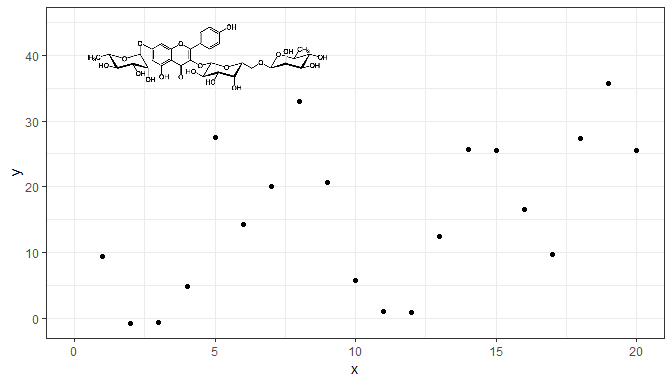Alternatively we produce the same plot by creating a data frame to contain the grob and the coordinates, and then map these variable to the aesthetics using aes().

logo.tb <- tibble(x = 0.02,
y = 0.95,
width = 2/3,
height = 1/4,
grob = list(grid::rasterGrob(image = Robinin)))

ggplot(data.tb, aes(x, y)) +
geom_grob_npc(data = logo.tb,
aes(label = grob, npcx = x, npcy = y,
vp.width = width, vp.height = height)) +
geom_point() +
expand_limits(y = 55, x = 0)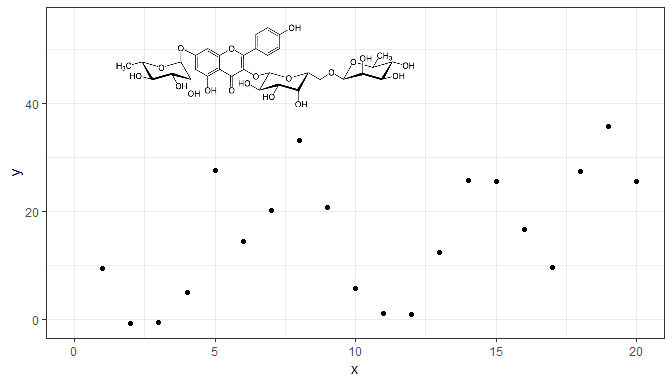Two geometries are based on existing ‘ggplot2’ geometries. They are almost like wrappers built on top of geom_text() and geom_label(). We give an example using geom_text_npc() to produce a “classic” labelling for facets matching the style of theme_classic() and traditional sicentific journals’ design.

corner_letters.tb <- tibble(label = LETTERS[1:4],
x = "left",
y = "top",
cyl = c(4,5,6,8))
ggplot(mpg, aes(displ, hwy)) +
geom_point() +
facet_wrap(~cyl, scales = "free") +
geom_text_npc(data = corner_letters.tb,
aes(npcx = x, npcy = y, label = label)) +
theme_classic() +
theme(strip.background = element_blank(),
strip.text.x = element_blank())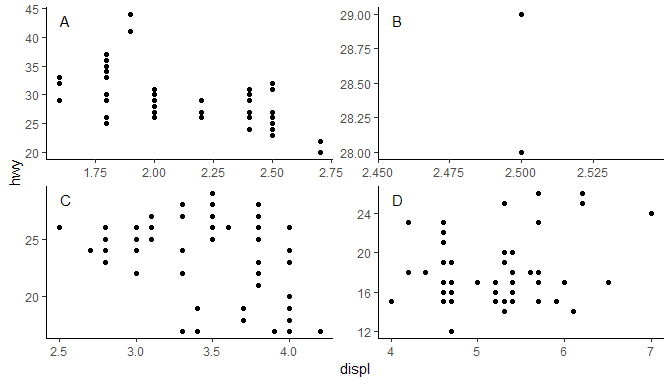Marginal markings

‘ggplot2’ provides geom_rug(), geom_vline() and geom_hline(). Rug plots are intended to be used to represent distributions along the margins of plot. geom_vline() and geom_hline()are normally used to separate regions in a plot or to highlight important values along the x or y axis. When creating plots it is sometimes useful to put small marks along the axes, just inside the plotting area, similar to those in a rug plot, but like geom_vline() and geom_hline() in their purpose.

Three geometries provide such markers: geom_margin_point(), geom_margin_arrow(), and geom_margin_grob(). They behave similarly to geom_vline() and geom_hline() and their positiions are determined also by the xintercept and yintercept aesthetics.

In the example below we indicate the group medians along the x axis with filled triangles.

data.tb <- mpg %>%
group_by(cyl) %>%
summarise(hwy = median(hwy), displ = median(displ))
ggplot(mpg, aes(displ, hwy, colour = factor(cyl))) +
geom_x_margin_point(data = data.tb,
aes(xintercept = displ, fill = factor(cyl))) +
expand_limits(y = 10) +
geom_point()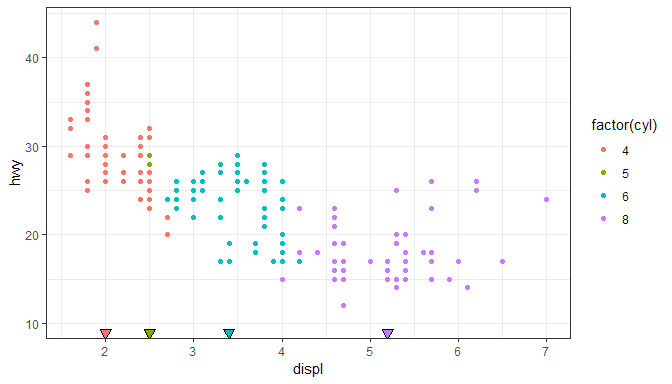stat_peaks and stat_valleys

Two statistics make it possible to highlight and/or label peaks and valleys (local maxima and local minima) in a curve. Here we use a time series, using POSIXct for time and the default formatting of labels. We also instruct geom_text() not to render overlapping labels.

ggplot(lynx, as.numeric = FALSE) + geom_line() +
stat_peaks(colour = "red") +
stat_peaks(geom = "text", colour = "red", vjust = -0.5,
check_overlap = TRUE) +
ylim(-100, 7300)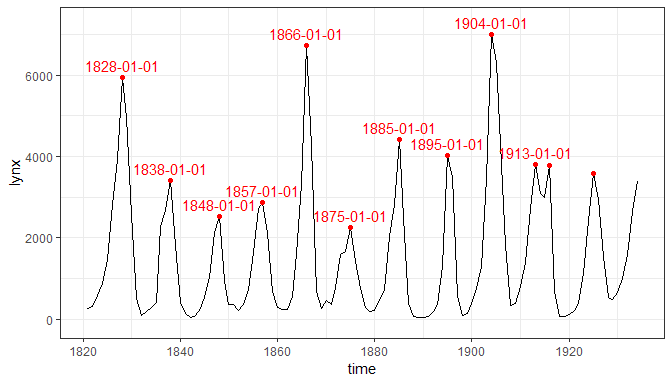Using numeric values for time and the default formatting of labels. We can also pass other aesthetics recognised the geom_text() such as angle. Here we also highlight and label the valleys.

ggplot(lynx) + geom_line() +
stat_peaks(colour = "red") +
stat_peaks(geom = "text", colour = "red", hjust = -0.2, vjust = 0.5,
angle = 90, check_overlap = TRUE) +
stat_valleys(colour = "blue") +
stat_valleys(geom = "text", colour = "blue", hjust = 1.2, vjust = 0.5,
angle = 90, check_overlap = TRUE) +
expand_limits(y = c(-900, 8000))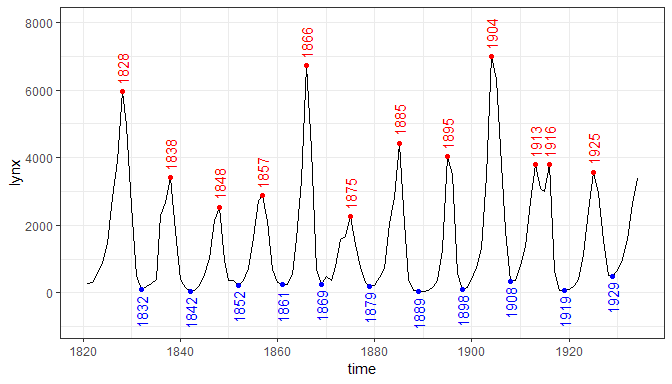Using POSIXct for time but supplying a format string. In addition marking both peaks and valleys.

ggplot(lynx, as.numeric = FALSE) + geom_line() +
stat_peaks(colour = "red") +
stat_peaks(geom = "text", colour = "red", hjust = -0.2, vjust = 0.5,
angle = 90, check_overlap = TRUE, x.label.fmt = "%Y") +
stat_valleys(colour = "blue") +
stat_valleys(geom = "text", colour = "blue", hjust = 1.2, vjust = 0.5,
angle = 90, check_overlap = TRUE, x.label.fmt = "%Y") +
expand_limits(y = c(-900, 8000))Using geom_rug for the peaks and valleys.

ggplot(lynx, as.numeric = FALSE) + geom_line() +
stat_peaks(colour = "red") +
stat_peaks(geom = "rug", colour = "red") +
stat_valleys(colour = "blue") +
stat_valleys(geom = "rug", colour = "blue")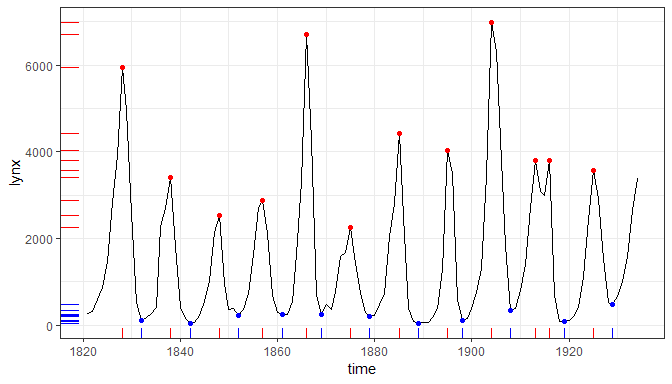This statistic automates the annotation of plots with number of observations, either by quadrant, by pairs of quadrants or the four quadrants taken together (whole plotting area).

We generate some artificial data.

set.seed(4321)
# generate artificial data
x <- -99:100
y <- x + rnorm(length(x), mean = 0, sd = abs(x))
my.data <- data.frame(x,
y,
group = c("A", "B"))

Using defaults except for colour.

ggplot(my.data, aes(x, y)) +
geom_hline(yintercept = 0, colour = "red") +
geom_vline(xintercept = 0, colour = "red") +
geom_point() +
expand_limits(y = c(-250, 250)) +
stat_quadrant_counts(colour = "red")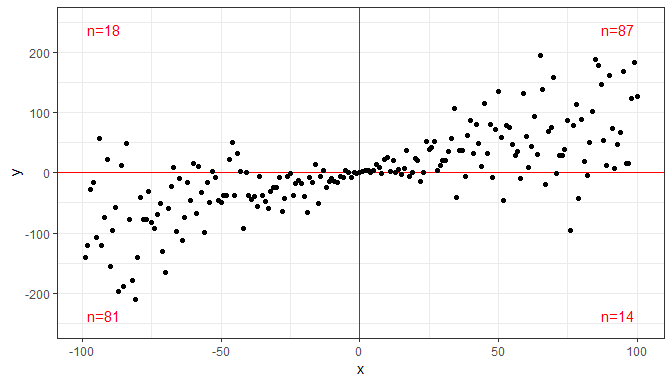Pooling quadrants along the x-axis. (pool.along = "y" pools along y.)

ggplot(my.data, aes(x, y)) +
geom_hline(yintercept = 0, colour = "red") +
geom_point() +
stat_quadrant_counts(colour = "red", pool.along = "x")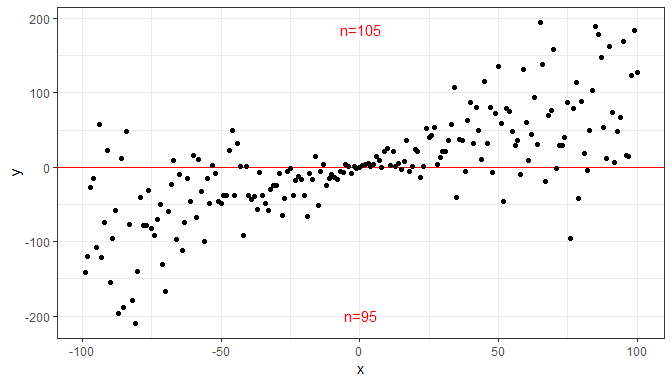Manual positioning of the text annotations and pooling of all four quadrants, and overriding the default formatting for the label.

ggplot(my.data, aes(x, y)) +
geom_point() +
aes(label = sprintf("%i observations", stat(count))))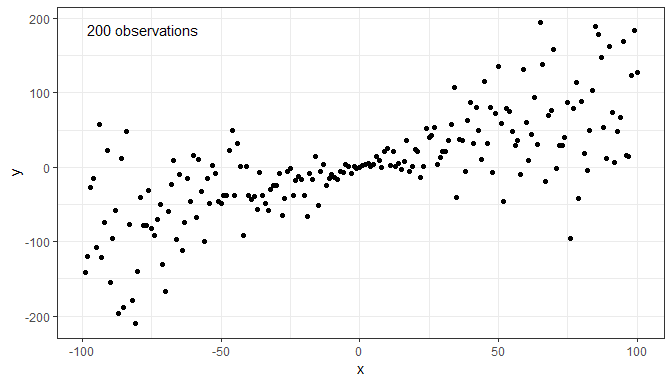ggplot(my.data, aes(x, y)) +
geom_hline(yintercept = 0, colour = "red") +
geom_vline(xintercept = 0, colour = "red") +
geom_point() +
stat_quadrant_counts(colour = "red", quadrants = c(2, 4))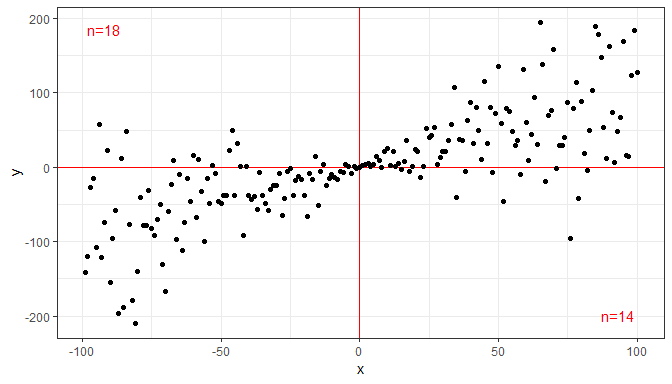Using facets, even with free scale limits, the labels are placed consistently. This achieved by the default use of geom_text_npc() or as shown below by use of geom_label_npc(). We expand the y limits to ensure that no observations are ocluded by the labels.

ggplot(my.data, aes(x, y, colour = group)) +
geom_hline(yintercept = 0) +
geom_vline(xintercept = 0) +
geom_point() +
stat_quadrant_counts(hjust = "inward", geom = "label_npc") +
expand_limits(y = c(-260, 260)) +
facet_wrap(~group)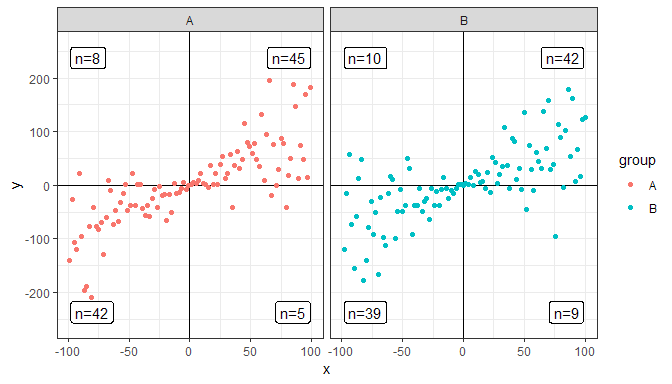stat_poly_eq

We generate another set of artificial data.

set.seed(4321)
# generate artificial data
x <- 1:100
y <- (x + x^2 + x^3) + rnorm(length(x), mean = 0, sd = mean(x^3) / 4)
my.data <- data.frame(x,
y,
group = c("A", "B"),
y2 = y * c(0.5,2),
block = c("a", "a", "b", "b"),
wt = sqrt(x))

First one example using defaults. To ensure that the formula used in both stats is the same, we first save it to a variable.

formula <- y ~ poly(x, 3, raw = TRUE)
ggplot(my.data, aes(x, y)) +
geom_point() +
stat_smooth(method = "lm", formula = formula) +
stat_poly_eq(formula = formula, parse = TRUE)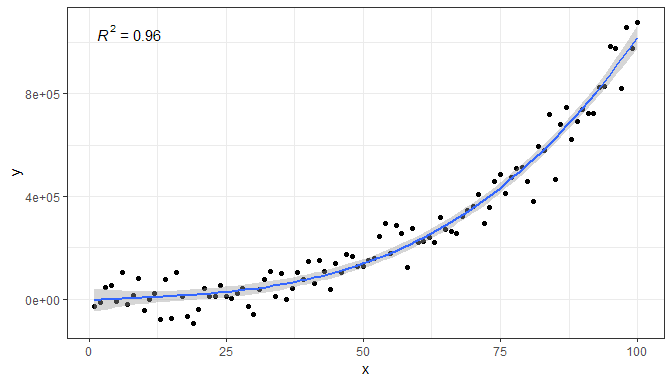stat_poly_eq() makes available three different labels in the returned data frame. One of these is used by default, but aes() can be used to map a different one to the label aesthetic. Here we show the adjusted coefficient of determination and the two information criteria.

formula <- y ~ poly(x, 3, raw = TRUE)
ggplot(my.data, aes(x, y)) +
geom_point() +
geom_smooth(method = "lm", formula = formula) +
stat_poly_eq(aes(label = ..adj.rr.label..), formula = formula,
parse = TRUE) +
stat_poly_eq(aes(label = ..AIC.label..),
label.x = "right", label.y = "bottom", size = 3,
formula = formula,
parse = TRUE)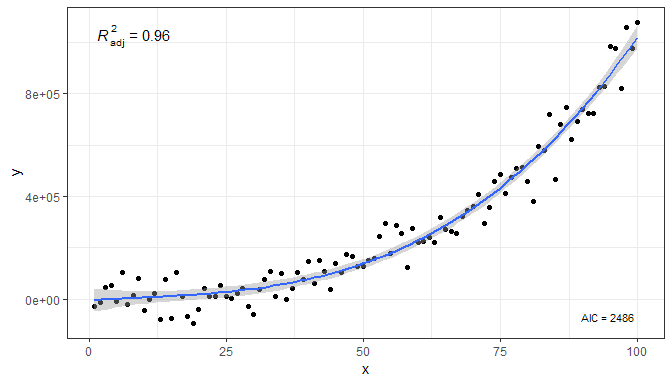A ready formatted equation is also returned as a string that needs to be parsed into an experession for display.

formula <- y ~ poly(x, 3, raw = TRUE)
ggplot(my.data, aes(x, y)) +
geom_point() +
geom_smooth(method = "lm", formula = formula) +
stat_poly_eq(aes(label = ..eq.label..), formula = formula,
parse = TRUE)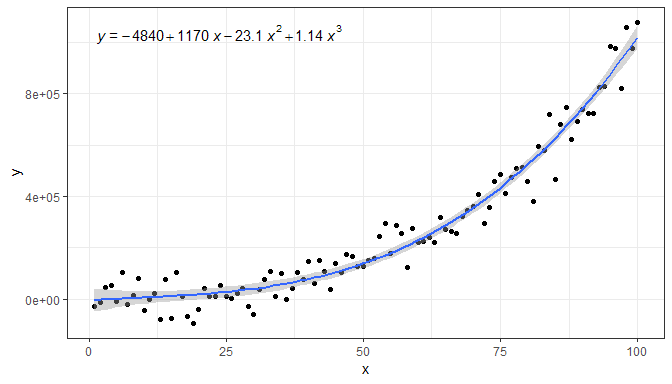Within aes() it is possible to compute new labels based on those returned plus “arbitrary” text. The supplied labels are meant to be parsed into expressions, so any text added should be valid for a string that will be parsed.

formula <- y ~ poly(x, 3, raw = TRUE)
ggplot(my.data, aes(x, y)) +
geom_point() +
geom_smooth(method = "lm", formula = formula) +
stat_poly_eq(aes(label =  paste(..eq.label.., ..adj.rr.label.., sep = "~~~~~~")),
formula = formula, parse = TRUE)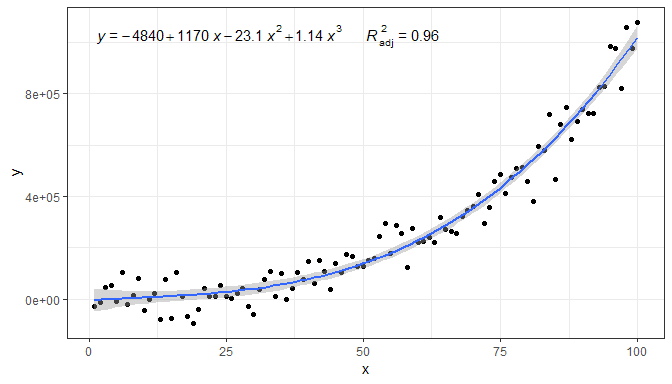Above we inserted spaces between the labels, but it is possible to add also other characters, even character strings. In this example we use several labels instead of just two, and separate them with comma followed by a space. Do note the need to escape the embedded quotation marks and to use plotmath trickery to add the comma at the end of the equation. We also change the size of the text in the label.

formula <- y ~ poly(x, 3, raw = TRUE)
ggplot(my.data, aes(x, y)) +
geom_point() +
geom_smooth(method = "lm", formula = formula) +
stat_poly_eq(aes(label =  paste(..eq.label.., ..rr.label..,
..AIC.label.., ..BIC.label..,
sep = "*\",\"~~")),
formula = formula, parse = TRUE, size = 3.5)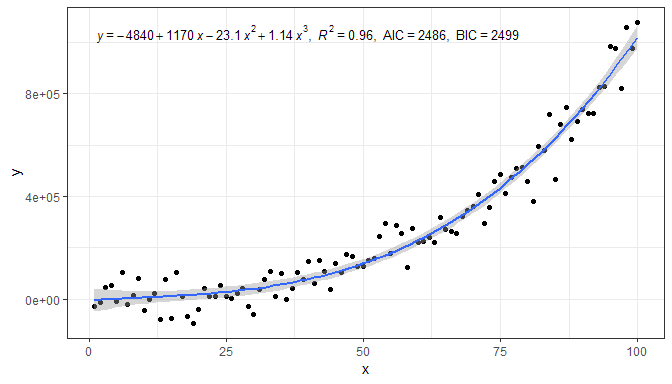It is also possible to insert arbitrary text, and format it, using plain(), italic(), bold() or bolditalic() as described in plotmath.

formula <- y ~ poly(x, 3, raw = TRUE)
ggplot(my.data, aes(x, y)) +
geom_point() +
geom_smooth(method = "lm", formula = formula) +
sep = "~~italic(\"with\")~~")),
formula = formula, parse = TRUE)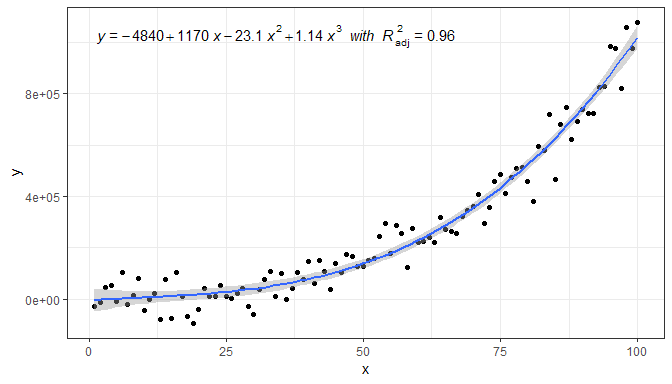As these are expressions, to include two lines of text, we need eithe to add stat_poly_eq() twice, passing an argument to label.y to reposition one of the labels (not shown) or use (as shown) atop() within the experssion to create a single label with two lines of text.

formula <- y ~ poly(x, 3, raw = TRUE)
ggplot(my.data, aes(x, y)) +
geom_point() +
geom_smooth(method = "lm", formula = formula) +
stat_poly_eq(aes(label = paste("atop(", ..AIC.label.., ",", ..BIC.label.., ")", sep = "")),
formula = formula,
parse = TRUE)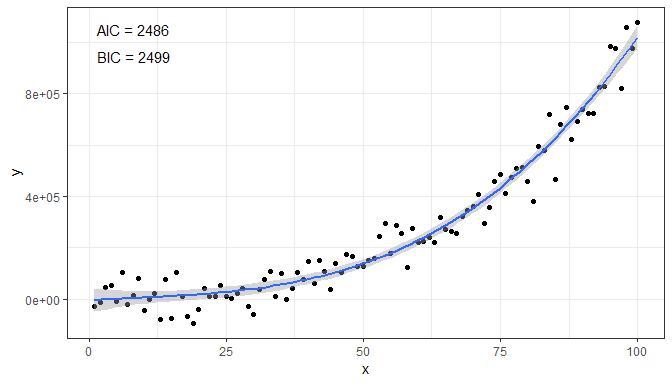One example removing the left hand side (lhs).

formula <- y ~ poly(x, 3, raw = TRUE)
ggplot(my.data, aes(x, y)) +
geom_point() +
geom_smooth(method = "lm", formula = formula) +
stat_poly_eq(aes(label = ..eq.label..),
eq.with.lhs = FALSE,
formula = formula, parse = TRUE)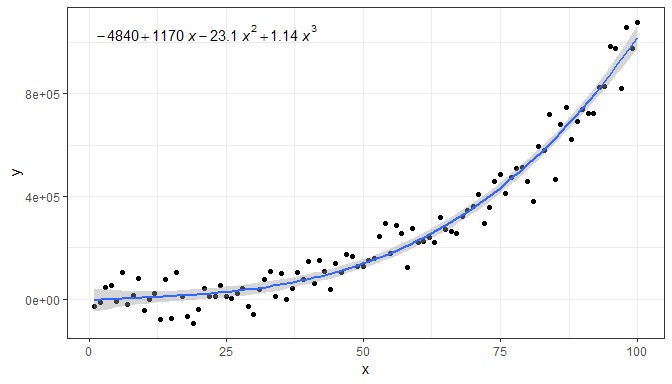Replacing the lhs.

formula <- y ~ poly(x, 3, raw = TRUE)
ggplot(my.data, aes(x, y)) +
geom_point() +
geom_smooth(method = "lm", formula = formula) +
stat_poly_eq(aes(label = ..eq.label..),
eq.with.lhs = "italic(hat(y))~=~",
formula = formula, parse = TRUE)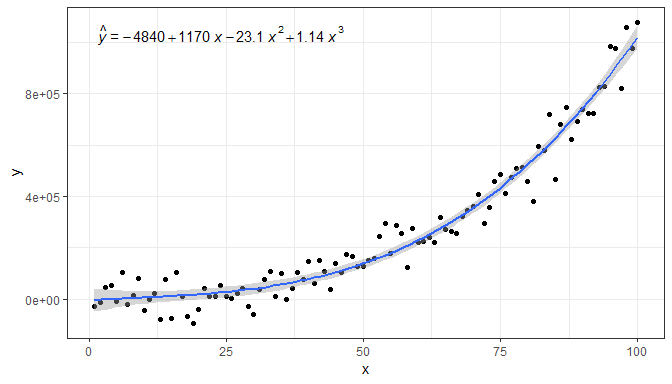And a final explample to replaced lhs and the variable symbol on the rhs.

formula <- y ~ poly(x, 3, raw = TRUE)
ggplot(my.data, aes(x, y)) +
geom_point() +
geom_smooth(method = "lm", formula = formula) +
labs(x = expression(italic(z)), y = expression(italic(h)) ) +
stat_poly_eq(aes(label = ..eq.label..),
eq.with.lhs = "italic(h)~=~",
eq.x.rhs = "~italic(z)",
formula = formula, parse = TRUE)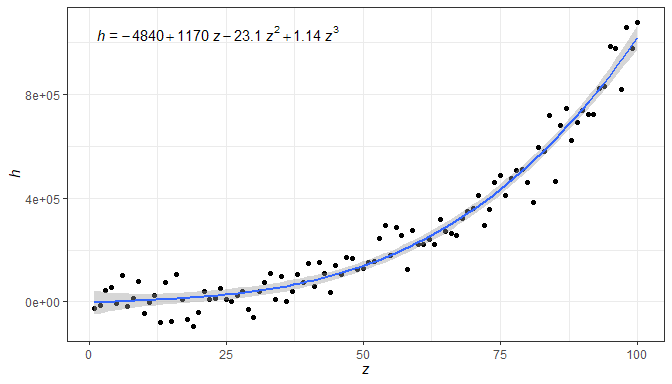As any valid R expression can be used, Greek letters are also supported, as well as the inclusion in the label of variable transformations used in the model formula.

formula <- y ~ poly(x, 2, raw = TRUE)
ggplot(my.data, aes(x, log10(y + 1e6))) +
geom_point() +
geom_smooth(method = "lm", formula = formula) +
stat_poly_eq(aes(label = ..eq.label..),
eq.with.lhs = "plain(log)(italic(y)+10^6)~=~",
formula = formula, parse = TRUE)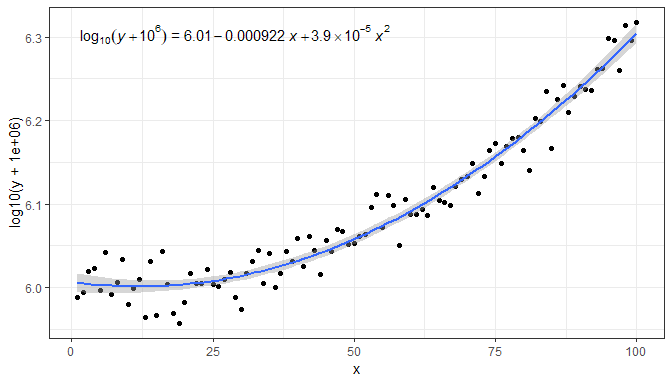A couple of additional examples of polynomials of different orders, and specified in different ways.

Higher order polynomial.

formula <- y ~ poly(x, 5, raw = TRUE)
ggplot(my.data, aes(x, y)) +
geom_point() +
geom_smooth(method = "lm", formula = formula) +
stat_poly_eq(aes(label = ..eq.label..), formula = formula, parse = TRUE)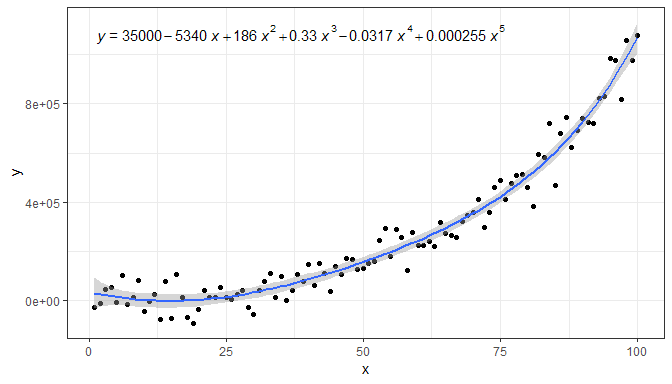Intercept forced to zero.

formula <- y ~ x + I(x^2) + I(x^3) - 1
ggplot(my.data, aes(x, y)) +
geom_point() +
geom_smooth(method = "lm", formula = formula) +
stat_poly_eq(aes(label = ..eq.label..), formula = formula,
parse = TRUE)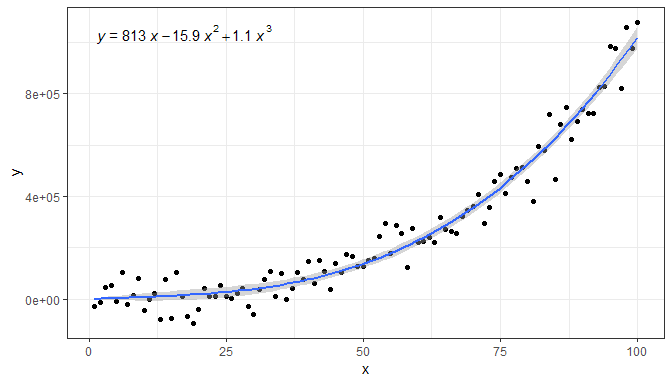We give below several examples to demonstrate how other components of the ggplot object affect the behaviour of this statistic.

Facets work as expected either with fixed or free scales. Although below we had to adjust the size of the font used for the equation.

formula <- y ~ poly(x, 3, raw = TRUE)
ggplot(my.data, aes(x, y2)) +
geom_point() +
geom_smooth(method = "lm", formula = formula) +
stat_poly_eq(aes(label = ..eq.label..), size = 3,
formula = formula, parse = TRUE) +
facet_wrap(~group)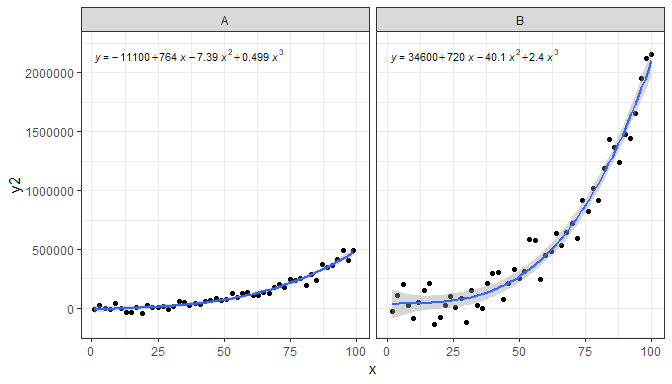formula <- y ~ poly(x, 3, raw = TRUE)
ggplot(my.data, aes(x, y2)) +
geom_point() +
geom_smooth(method = "lm", formula = formula) +
stat_poly_eq(aes(label = ..eq.label..), size = 3,
formula = formula, parse = TRUE) +
facet_wrap(~group, scales = "free_y")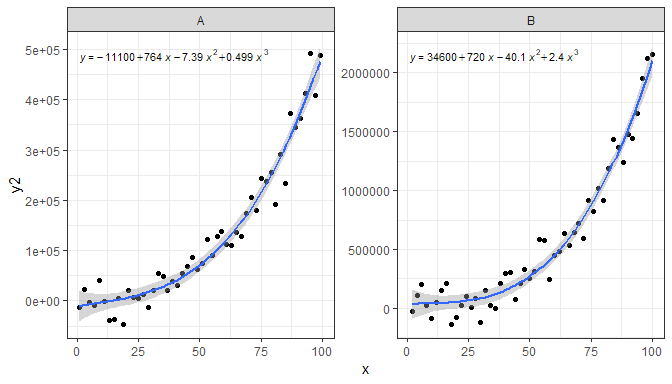Grouping, in this example using the colour aesthetic also works as expected. We can use justification and supply an absolute location for the equation, but the default frequently works well as in the example below.

formula <- y ~ poly(x, 3, raw = TRUE)
ggplot(my.data, aes(x, y2, colour = group)) +
geom_point() +
geom_smooth(method = "lm", formula = formula) +
stat_poly_eq(aes(label = ..eq.label..),
formula = formula, parse = TRUE)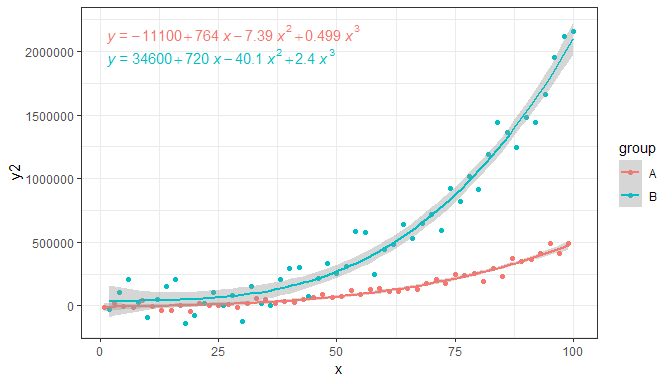Label positions relative to the ranges of the x and y scales are also supported, both through string constants and numeric values in the range 0 to 1, when using the default geom_text_npc().

formula <- y ~ poly(x, 3, raw = TRUE)
ggplot(my.data, aes(x, y2, colour = group)) +
geom_point() +
geom_smooth(method = "lm", formula = formula) +
stat_poly_eq(aes(label = ..eq.label..),
formula = formula, parse = TRUE,
label.x = "centre")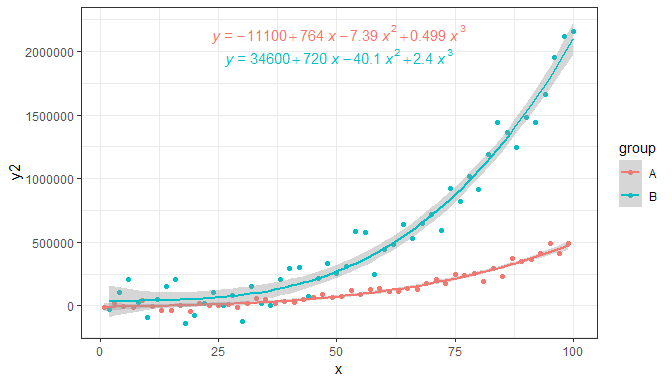The default locations are now based on normalized coordinates, and consequently these defaults work even when the range of the x and y scales varies from panel to panel.

formula <- y ~ poly(x, 3, raw = TRUE)
ggplot(my.data, aes(x, y2, fill = block)) +
geom_point(shape = 21, size = 3) +
geom_smooth(method = "lm", formula = formula) +
stat_poly_eq(aes(label = ..rr.label..), size = 3,
geom = "label_npc", alpha = 0.33,
formula = formula, parse = TRUE) +
facet_wrap(~group, scales = "free_y")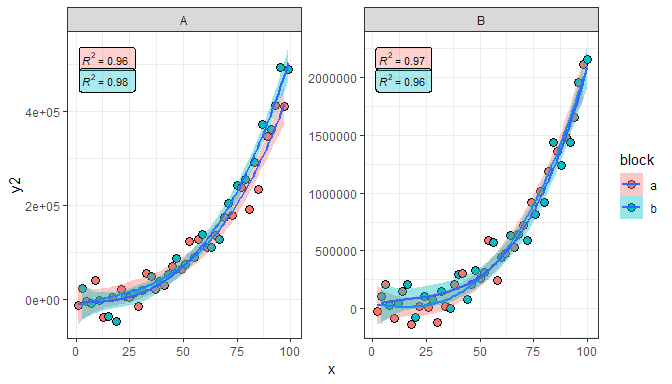At the moment if the same variable used for faceting is also mapped to an aesthetic the automatic location of labels results in “odd” alignment. This is bug that needs fixing.

formula <- y ~ poly(x, 3, raw = TRUE)
ggplot(my.data, aes(x, y2, colour = group, fill = block)) +
geom_point(shape = 21, size = 3) +
geom_smooth(method = "lm", formula = formula) +
stat_poly_eq(aes(label = ..rr.label..), size = 3,
geom = "label_npc", alpha = 0.2,
formula = formula, parse = TRUE) +
facet_wrap(~group, scales = "free_y")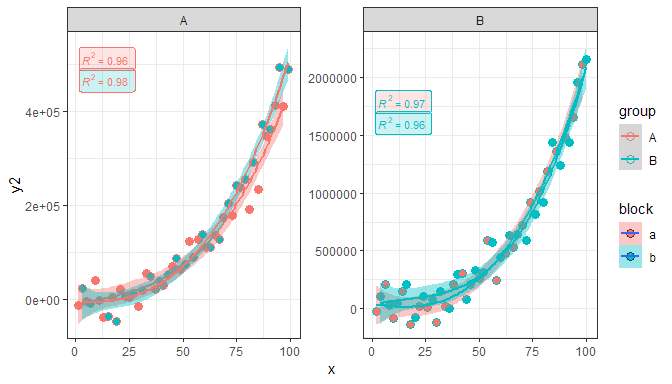It is possible to use geom_text() and geom_label() but in this case label coordinates need to be given explicitly in native data coordinates. When multiple labels need to be positioned a vector of coordinates can be used as shown here for label.y.

formula <- y ~ poly(x, 3, raw = TRUE)
ggplot(my.data, aes(x, y2, colour = group)) +
geom_point() +
geom_smooth(method = "lm", formula = formula) +
stat_poly_eq(geom = "text", aes(label = ..eq.label..),
label.x = 0, label.y = c(1e6, 1.2e6), hjust = 0,
formula = formula, parse = TRUE)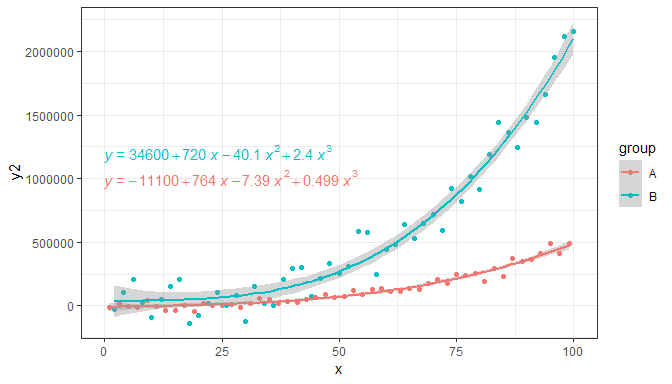stat_fit_residuals

I had the need to quickly plot residuals matching fits plotted with geom_smooth() using grouping and facets, so a new (simple) statistic was born.

formula <- y ~ poly(x, 3, raw = TRUE)
ggplot(my.data, aes(x, y, color = group)) +
geom_hline(yintercept = 0, linetype = "dashed") +
stat_fit_residuals(formula = formula)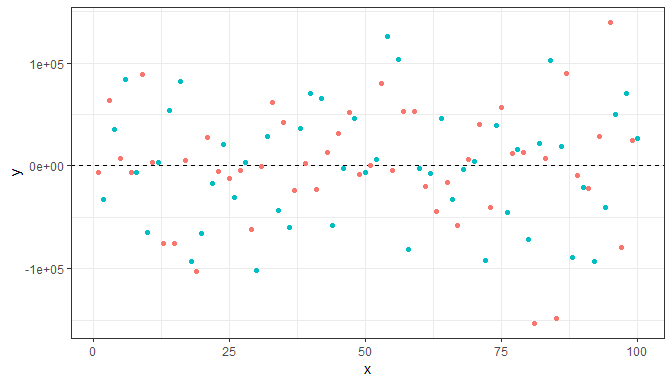stat_fit_deviations

As I also had the need to highlight residuals in slides and notes to be used in teaching, another statistic was born.

formula <- y ~ poly(x, 3, raw = TRUE)
ggplot(my.data, aes(x, y)) +
geom_smooth(method = "lm", formula = formula) +
stat_fit_deviations(formula = formula, color = "red") +
geom_point()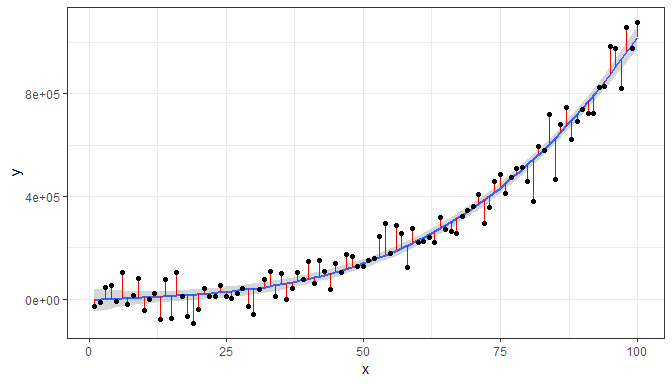The geometry used by default is geom_segment() to which additional aesthetics can be mapped. Here we add arrowheads.

formula <- y ~ poly(x, 3, raw = TRUE)
ggplot(my.data, aes(x, y)) +
geom_smooth(method = "lm", formula = formula) +
geom_point() +
stat_fit_deviations(formula = formula, color = "red",
arrow = arrow(length = unit(0.015, "npc"),
ends = "both"))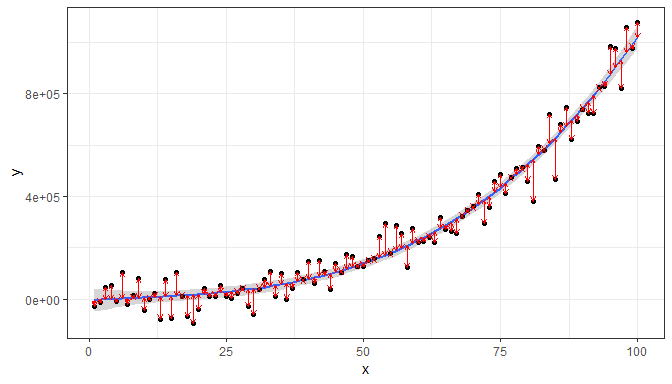stat_fit_glance

Package ‘broom’ provides consistently formated output from different model fitting functions. These makes possible to write model-annotation statistic that are very flexible put that require more effort with the definition of the character strings to map to the label aesthetic.

As we have above given some simple examples, we here exemplify this statistic in a plot with grouping, and assemble a label for the P-value using a string parsed into a expression. We also change the default position of the labels.

# formula <- y ~ poly(x, 3, raw = TRUE)
# broom::augment does not handle poly() correctly!
formula <- y ~ x + I(x^2) + I(x^3)
ggplot(my.data, aes(x, y, color = group)) +
geom_point() +
geom_smooth(method = "lm", formula = formula) +
stat_fit_glance(method = "lm",
method.args = list(formula = formula),
label.x = "right",
label.y = "bottom",
aes(label = paste("italic(P)*\"-value = \"*",
signif(..p.value.., digits = 4), sep = "")),
parse = TRUE)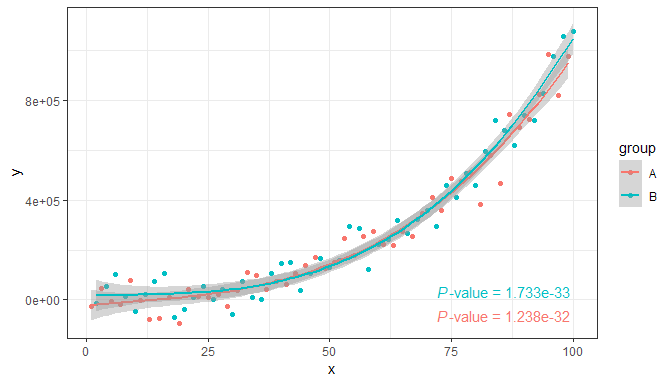It is also possible to fit a non-linear model with method = "nls", and any other model for which a glance() method exists. Do consult the documentation for package ‘broom’. Here we fit the Michaelis-Menten equation to reaction rate versus concentration data.

micmen.formula <- y ~ SSmicmen(x, Vm, K)
ggplot(Puromycin, aes(conc, rate, colour = state)) +
geom_point() +
geom_smooth(method = "nls",
formula = micmen.formula,
se = FALSE) +
stat_fit_glance(method = "nls",
method.args = list(formula = micmen.formula),
aes(label = paste("AIC = ", signif(..AIC.., digits = 3),
", BIC = ", signif(..BIC.., digits = 3),
sep = "")),
label.x = "centre", label.y = "bottom")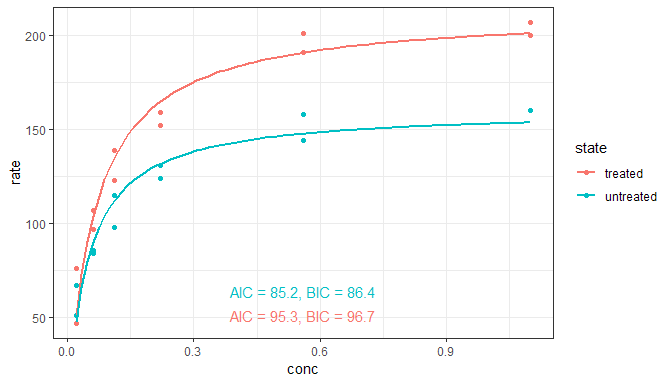stat_fit_tidy

This stat makes it possible to add the equation for any fitted model for which broom::tidy() is implemented. Alternatively, individual values such as estimates for the fitted parameters, standard errors, or P-values can be added to a plot. However, the user has to explicitly construct the labels within aes(). This statistic respects grouping based on aesthetics, and reshapes the output of tidy() so that the values for a given group are in a single row in the returned data.

micmen.formula <- y ~ SSmicmen(x, Vm, K)
ggplot(Puromycin, aes(conc, rate, colour = state)) +
geom_point() +
geom_smooth(method = "nls",
formula = micmen.formula,
se = FALSE) +
stat_fit_tidy(method = "nls",
method.args = list(formula = micmen.formula),
label.x = "right",
label.y = "bottom",
aes(label = paste("V[m]~=~", signif(..Vm_estimate.., digits = 3),
"%+-%", signif(..Vm_se.., digits = 2),
"~~~~K~=~", signif(..K_estimate.., digits = 3),
"%+-%", signif(..K_se.., digits = 2),
sep = "")),
parse = TRUE)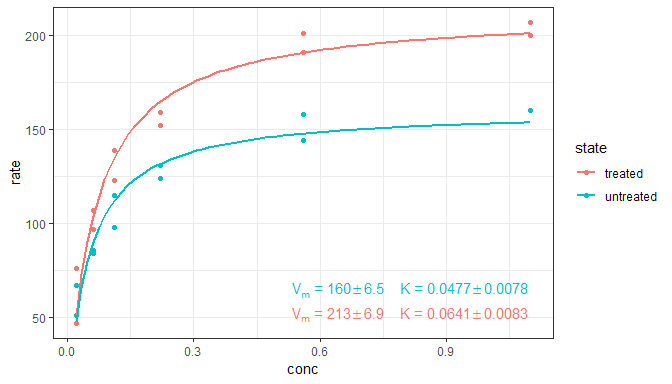micmen.formula <- y ~ SSmicmen(x, Vm, K)
ggplot(Puromycin, aes(conc, rate, colour = state)) +
geom_point() +
geom_smooth(method = "nls",
formula = micmen.formula,
se = FALSE) +
stat_fit_tidy(method = "nls",
method.args = list(formula = micmen.formula),
size = 3,
label.x = "center",
label.y = "bottom",
vstep = 0.18,
aes(label = paste("V~=~frac(", signif(..Vm_estimate.., digits = 2), "~C,",
signif(..K_estimate.., digits = 2), "+C)",
sep = "")),
parse = TRUE) +
labs(x = "C", y = "V")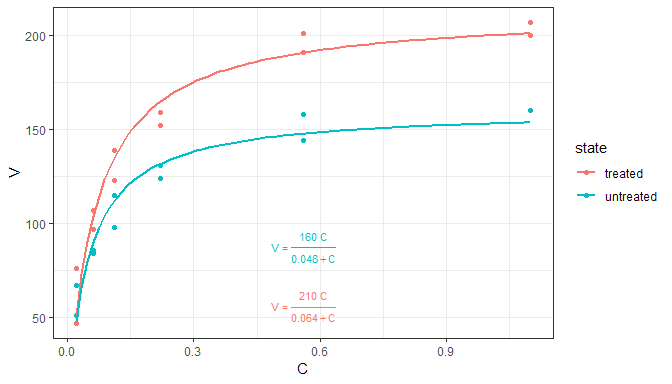stat_fit_tb

This stat makes it possible to add summary or ANOVA tables for any fitted model for which broom::tidy() is implemented. The output from tidy() is embedded as a single list value within the returned data, an object of class tibble. This statistic ignores grouping based on aesthetics. This allows fitting models when x or y is a factor (as in such cases ggplot splits the data into groups, one for each level of the factor, which is needed for example for stat_summary() to work as expected). By default, the "table" geometry is used. The use of geom_table() is described in a separate section of this User Guide.

The default output of stat_fit_tb is the default output from tidy(mf) where mf is the fitted model.

formula <- y ~ x + I(x^2) + I(x^3)
ggplot(my.data, aes(x, y)) +
geom_point() +
geom_smooth(method = "lm", formula = formula) +
stat_fit_tb(method = "lm",
method.args = list(formula = formula),
tb.vars = c(Parameter = "term",
Estimate = "estimate",
"s.e." = "std.error",
"italic(t)" = "statistic",
"italic(P)" = "p.value"),
label.y = "top", label.x = "left",
parse = TRUE)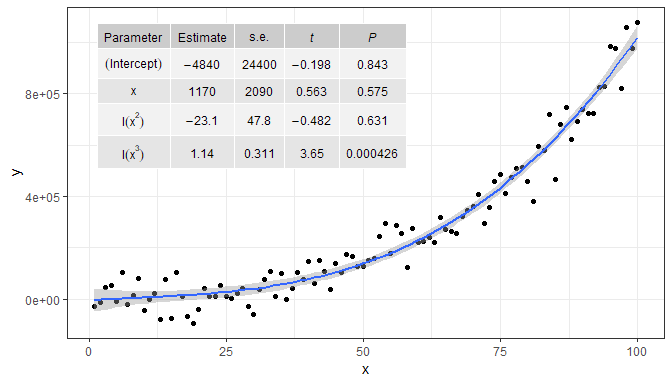When tb.type = "fit.anova" the output returned is that from tidy(anova(mf)) where mf is the fitted model.

formula <- y ~ x + I(x^2) + I(x^3)
ggplot(my.data, aes(x, y)) +
geom_point() +
geom_smooth(method = "lm", formula = formula) +
stat_fit_tb(method = "lm",
method.args = list(formula = formula),
tb.type = "fit.anova",
tb.vars = c(Effect = "term",
"df",
"M.S." = "meansq",
"italic(F)" = "statistic",
"italic(P)" = "p.value"),
label.y = "top", label.x = "left",
parse = TRUE)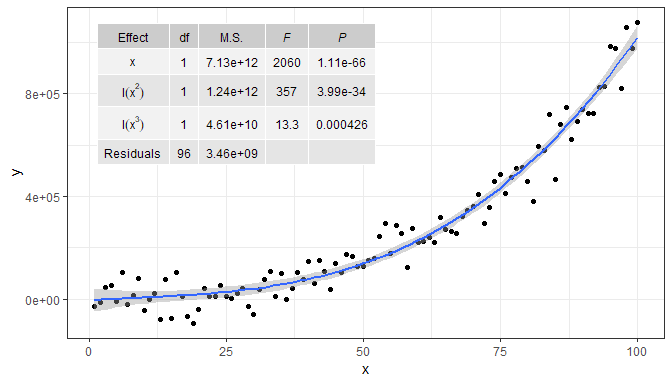When tb.type = "fit.coefs" the output returned is that of tidy(mf) after selecting the term and estimate columns.

formula <- y ~ x + I(x^2) + I(x^3)
ggplot(my.data, aes(x, y)) +
geom_point() +
geom_smooth(method = "lm", formula = formula) +
stat_fit_tb(method = "lm",
method.args = list(formula = formula),
tb.type = "fit.coefs",
label.y = "center", label.x = "left")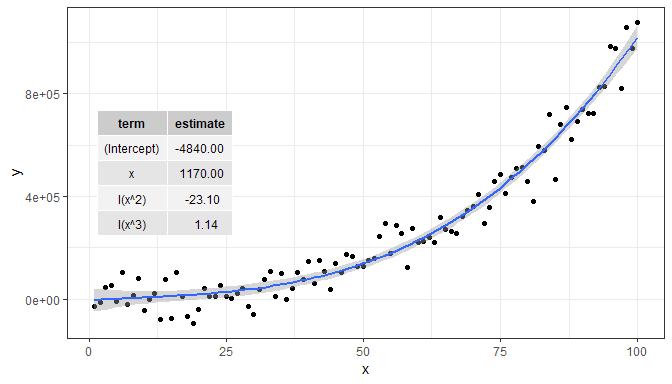Faceting works as expected, but grouping is ignored as mentioned above. In this case, the colour aesthetic is not applied to the text of the tables. Furthermore, if label.x.npc or label.y.npc are passed numeric vectors of length > 1, the values are obeyed by the different panels.

micmen.formula <- y ~ SSmicmen(x, Vm, K)
ggplot(Puromycin, aes(conc, rate, colour = state)) +
facet_wrap(~state) +
geom_point() +
geom_smooth(method = "nls",
formula = micmen.formula,
se = FALSE) +
stat_fit_tb(method = "nls",
method.args = list(formula = micmen.formula),
tb.type = "fit.coefs",
label.x = 0.9,
label.y = c(0.75, 0.2)) +
theme(legend.position = "none") +
labs(x = "C", y = "V")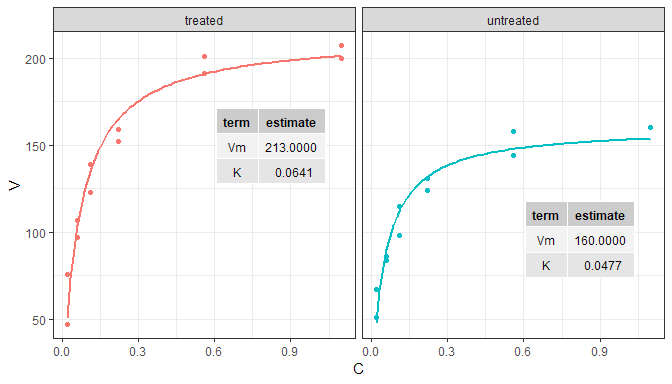The data in the example below are split by ggplot into six groups based on the levels of the feed factor. However, as stat_fit_tb() ignores groupings, we can still fit a linear model to all the data in the panel.

ggplot(chickwts, aes(factor(feed), weight)) +
stat_summary(fun.data = "mean_se") +
stat_fit_tb(tb.type = "fit.anova",
label.x = "center",
label.y = "bottom") +
expand_limits(y = 0)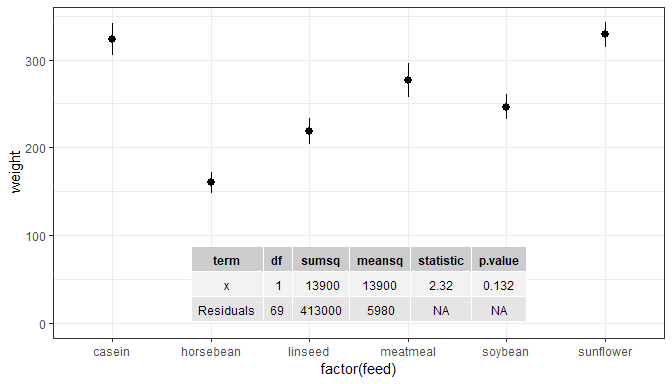We can flip the system of coordinates, if desired.

ggplot(chickwts, aes(factor(feed), weight)) +
stat_summary(fun.data = "mean_se") +
stat_fit_tb(tb.type = "fit.anova", label.x = "left", size = 3) +
scale_x_discrete(expand = expand_scale(mult = c(0.2, 0.5))) +
coord_flip()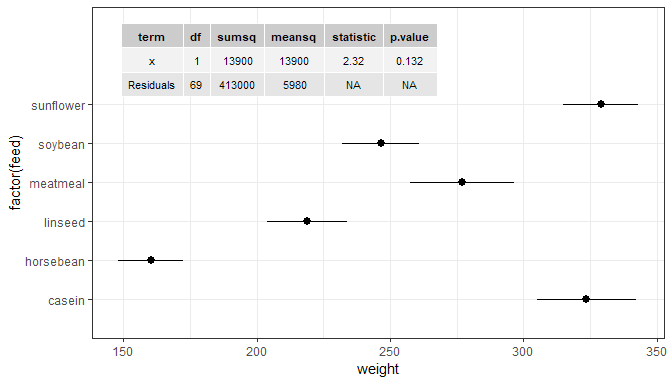It is also possible to rotate the table using angle.

ggplot(chickwts, aes(factor(feed), weight)) +
stat_summary(fun.data = "mean_se") +
stat_fit_tb(tb.type = "fit.anova",
angle = 90, size = 3,
label.x = "right", label.y = "center",
hjust = 0.5, vjust = 0,
tb.vars = c(Effect = "term",
"df",
"M.S." = "meansq",
"italic(F)" = "statistic",
"italic(P)" = "p.value"),
parse = TRUE) +
scale_x_discrete(expand = expand_scale(mult = c(0.1, 0.35))) +
expand_limits(y = 0)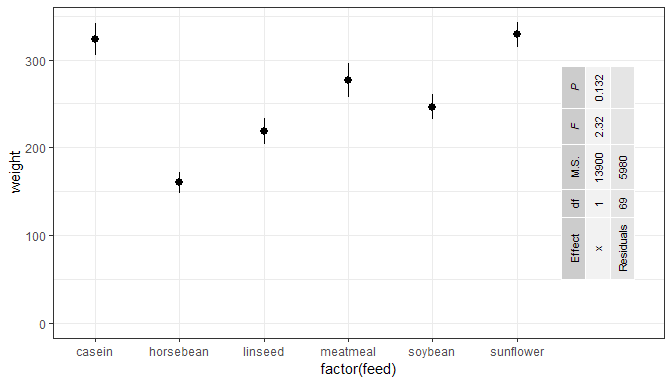stat_fit_augment

Experimental! Use ggplot2::stat_smooth instead of stat_fit_augment if possible.

For a single panel and no grouping, there is little advantage in using this statistic compared to the examples in the documentation of package ‘broom’. With grouping and faceting stat_fit_augment may occasionally be more convenient than ggplot2::stat_smooth because of its flexibility.

# formula <- y ~ poly(x, 3, raw = TRUE)
# broom::augment does not handle poly correctly!
formula <- y ~ x + I(x^2) + I(x^3)
ggplot(my.data, aes(x, y)) +
geom_point() +
stat_fit_augment(method = "lm",
method.args = list(formula = formula))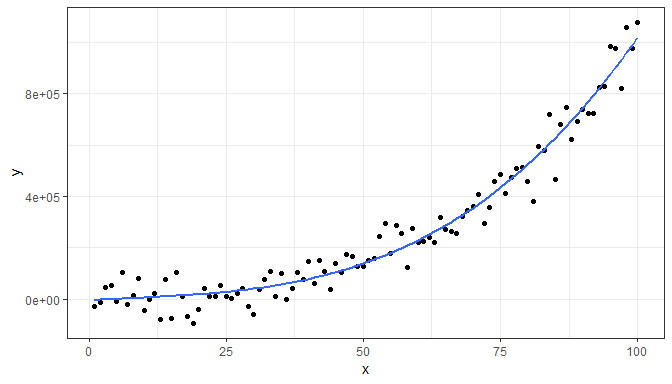formula <- y ~ x + I(x^2) + I(x^3)
ggplot(my.data, aes(x, y, color = group)) +
geom_point() +
stat_fit_augment(method = "lm",
method.args = list(formula = formula))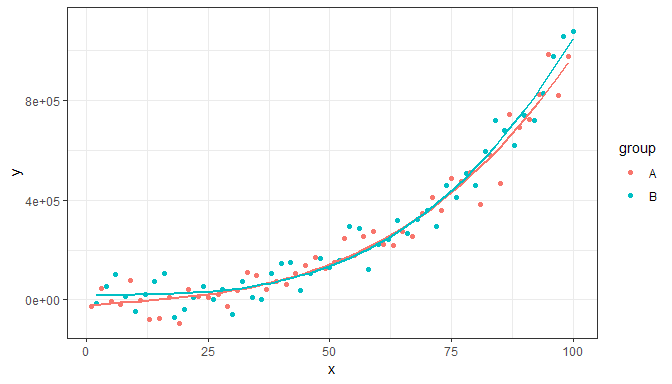We can override the variable returned as y to be any of the variables in the data frame returned by broom::augment while still preserving the original y values.

formula <- y ~ x + I(x^2) + I(x^3)
ggplot(my.data, aes(x, y)) +
stat_fit_augment(method = "lm",
method.args = list(formula = formula),
geom = "point",
y.out = ".resid")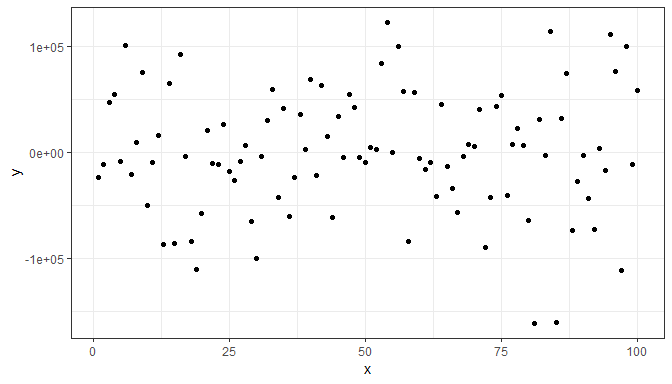formula <- y ~ x + I(x^2) + I(x^3)
ggplot(my.data, aes(x, y, color = group)) +
stat_fit_augment(method = "lm",
method.args = list(formula = formula),
geom = "point",
y.out = ".std.resid")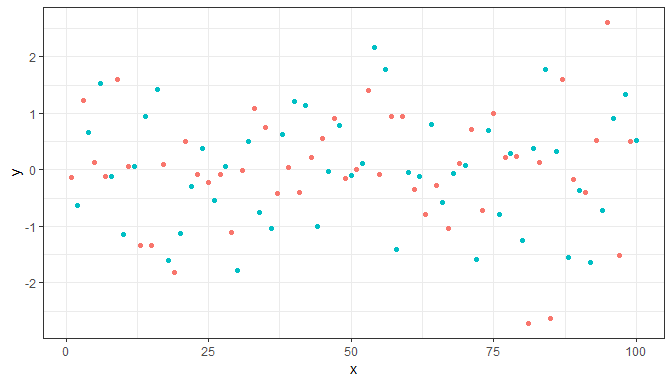We can use any model fitting method for which augment is implemented.

args <- list(formula = y ~ k * e ^ x,
start = list(k = 1, e = 2))
ggplot(mtcars, aes(wt, mpg)) +
geom_point() +
stat_fit_augment(method = "nls",
method.args = args)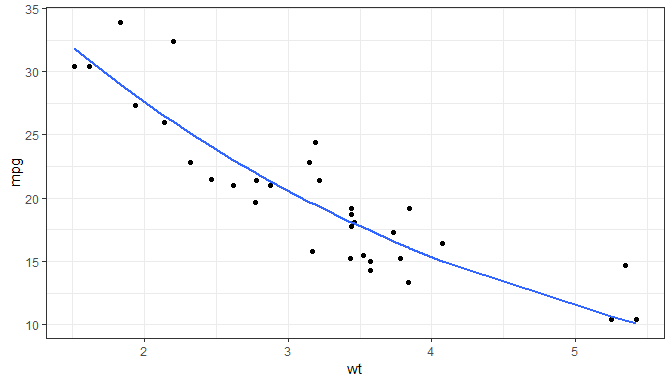args <- list(formula = y ~ k * e ^ x,
start = list(k = 1, e = 2))
ggplot(mtcars, aes(wt, mpg)) +
stat_fit_augment(method = "nls",
method.args = args,
geom = "point",
y.out = ".resid")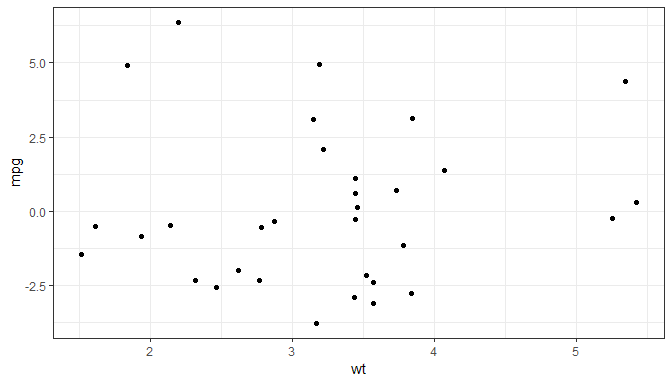args <- list(model = y ~ SSlogis(x, Asym, xmid, scal),
fixed = Asym + xmid + scal ~1,
random = Asym ~1 | group,
start = c(Asym = 200, xmid = 725, scal = 350))
ggplot(Orange, aes(age, circumference, color = Tree)) +
geom_point() +
stat_fit_augment(method = "nlme",
method.args = args,
augment.args = list(data = quote(data)))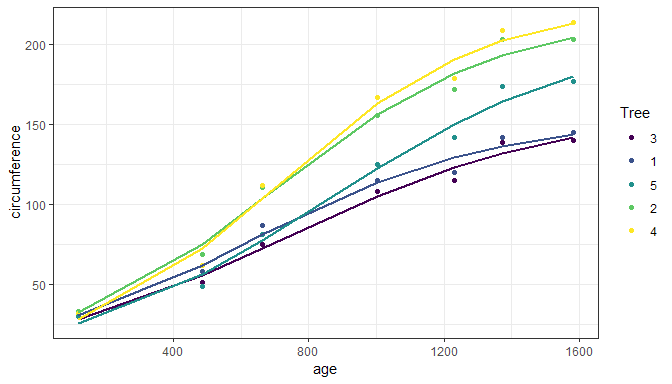stat_dens2d_labels and stat_dens2d_filter

These stats had their origin in an enhancement suggestion for ‘ggrepel’ from Hadley Wickham and discussion with Kamil Slowikowski (ggrepel’s author) and others. In fact the code is based on code Kamil gave during the discussion, but simplified and taking a few further ideas from ggplot::stat_dens2d.

Warning! Which observations are selected by the algorithm used, based on MASS:kde2d, depends strongly on the values of parameters h and n. You may need to alter the defaults by passing explicit arguments to these stats. Beware, though, that what are good values, may depend on individual data sets even if they include the same number of observations. For the selection of observations to work cleanly, the argument for n must create a dense grid. Much larger values of n than in the examples in the documentation of MASS::kde2d and ggplot2::stat_dens2d will be needed in most cases.

Some random data with random labels.

random_string <- function(len = 6) {
paste(sample(letters, len, replace = TRUE), collapse = "")
}

# Make random data.
set.seed(1001)
d <- tibble::tibble(
x = rnorm(100),
y = rnorm(100),
group = rep(c("A", "B"), c(50, 50)),
lab = replicate(100, { random_string() })
)

stat_dens2d_filter

The stat stat_dens2d_filter filters observations, in other words passes to the geom a subset of the data received as input. The default value for geom is "point".

Using defaults except for the color aesthetic. Highlight 1/10 of observations from lowest density areas of the plot panel.

ggplot(data = d, aes(x, y)) +
geom_point() +
stat_dens2d_filter(color = "red")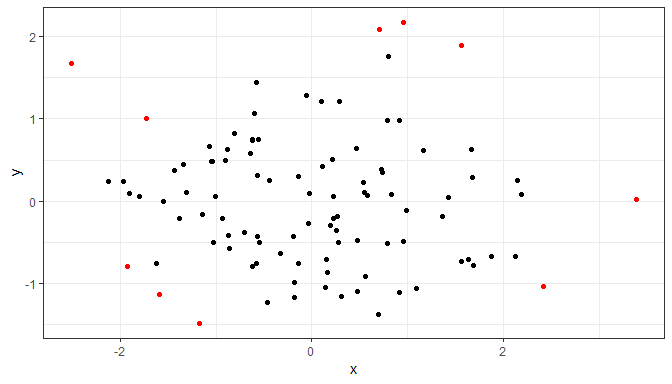Highlighting 1/4 of the observations by under-plotting with larger black points.

ggplot(data = d, aes(x, y, color = group)) +
stat_dens2d_filter(keep.fraction = 0.25,
size = 3,
color = "black") +
geom_point()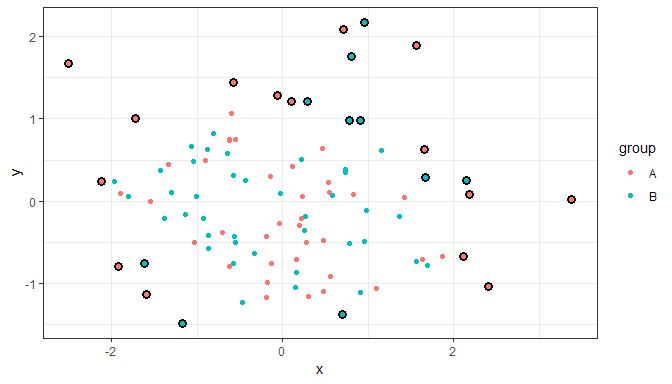A different way of highlighting 1/4 of the observations, using over-plotting with a ‘hollow’ shape. We also shift one group with respect to the other.

ggplot(data = d, aes(x + rep(c(-2,2), rep(50,2)),
y, color = group)) +
geom_point() +
stat_dens2d_filter(shape = 1, size = 3,
keep.fraction = 0.25)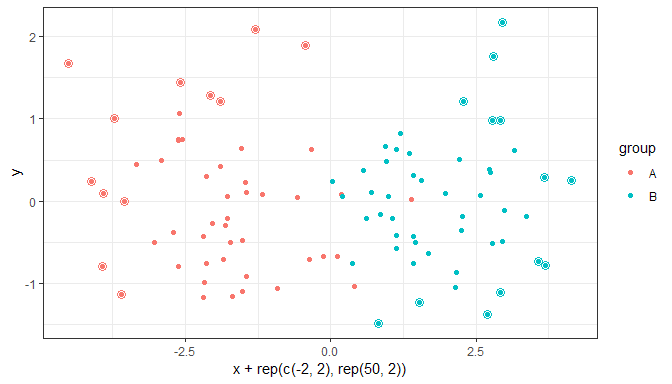Highlight 1/4 of observations from lowest density areas of the plot, with density considered separately for each individual group. In this case grouping is based on the color aesthetic.

ggplot(data = d, aes(x + rep(c(-2,2), rep(50,2)),
y, color = group)) +
geom_point() +
stat_dens2d_filter_g(shape = 1, size = 3,
keep.fraction = 0.25)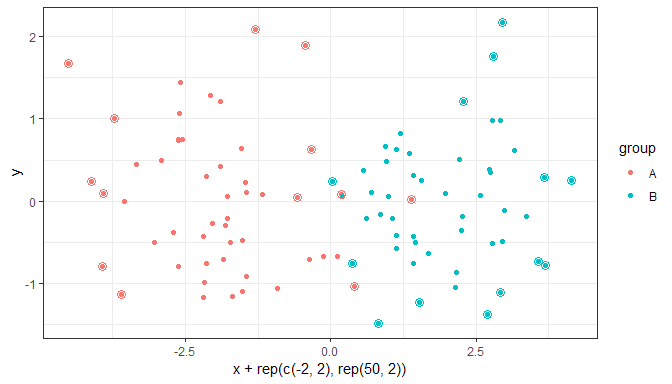Add text labels to 1/10 of the observations. The “text_repel” geom sees only these observations.

ggplot(data = d, aes(x, y, label = lab, color = group)) +
geom_point() +
stat_dens2d_filter(geom = "text_repel")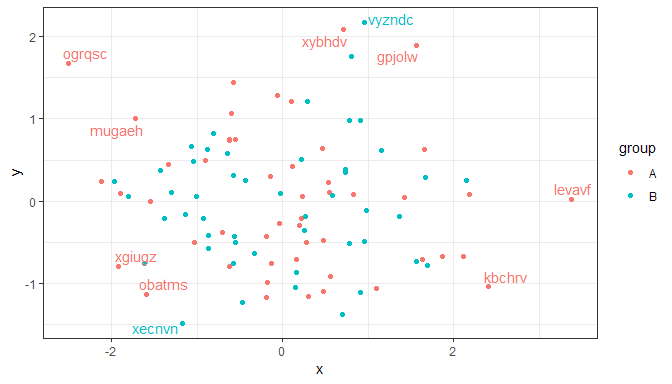Add text labels to 1/2 of the observations.

ggplot(data = d, aes(x, y, label = lab, color = group)) +
geom_point() +
stat_dens2d_filter(geom = "text_repel", keep.fraction = 0.5)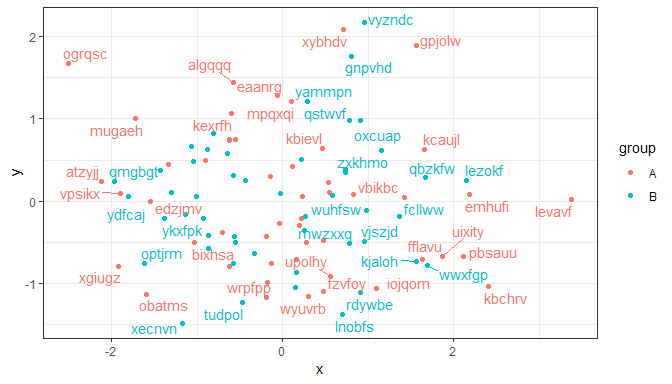stat_dens2d_labels

The stat stat_dens2d_labels replaces the values of the label (aesthetic) variable in data in the high density regions of the panel with the argument passed to label.fill before passing them to the geom. The default value for geom is "text". The default value of label.fill is "" which results in empty labels, while using NA as fill label results in observations being omitted. Using NA as label.fill is not too different from using stat_dens2d_filter as long as the geom used requires a label aesthetic.

Label 1/10 of observations from lowest density areas of the plot panels.

ggplot(data = d, aes(x, y, label = lab, color = group)) +
geom_point() +
stat_dens2d_labels()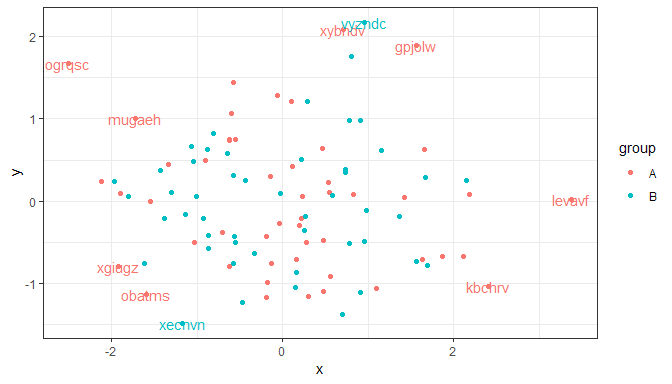Add text labels to 45% of the observations.

ggplot(data = d, aes(x, y, label = lab, color = group)) +
geom_point() +
stat_dens2d_labels(keep.fraction = 0.45)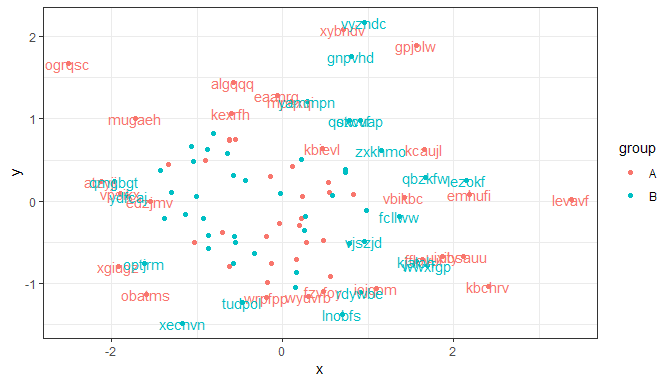When using geom "text" we can statically adjust the positioning of labels, but this is rarely enough even when keeping 1/4 of the labels.

ggplot(data = d, aes(x, y, label = lab, color = group)) +
geom_point() +
stat_dens2d_labels(keep.fraction = 0.25,
vjust = -0.3)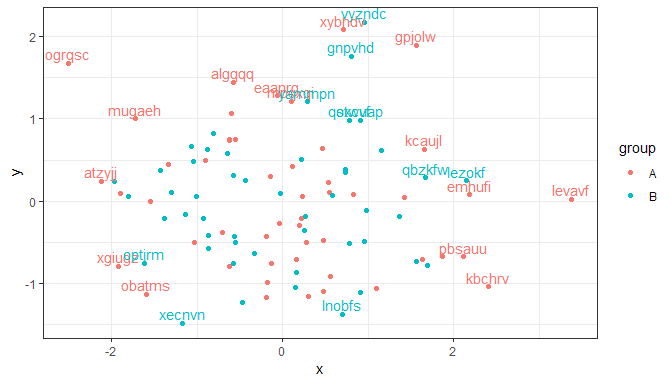Using the geoms from package ‘ggrepel’ avoids clashes among labels or on top of data points. This works with versions 0.6.0 and newer of ‘ggrepel’. One example with geom_text_repel follows.

ggplot(data = d, aes(x, y, label = lab, color = group)) +
geom_point() +
stat_dens2d_labels(geom = "text_repel",
keep.fraction = 0.45)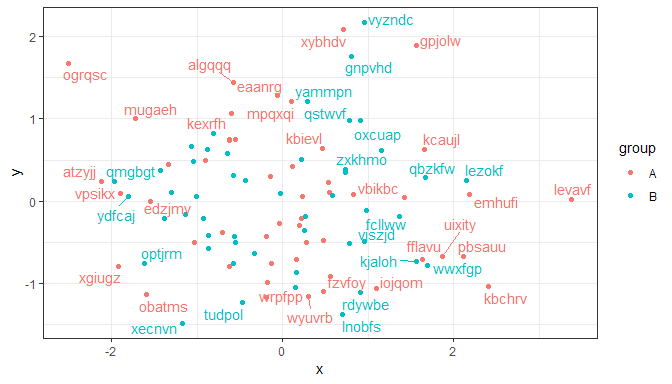With geom_label_repel one needs to use a smaller value for keep.fracton, or a smaller size, as labels use more space on the plot than the test alone.

ggplot(data = d, aes(x, y, label = lab, color = group)) +
geom_point() +
stat_dens2d_labels(geom = "label_repel",
keep.fraction = 0.25)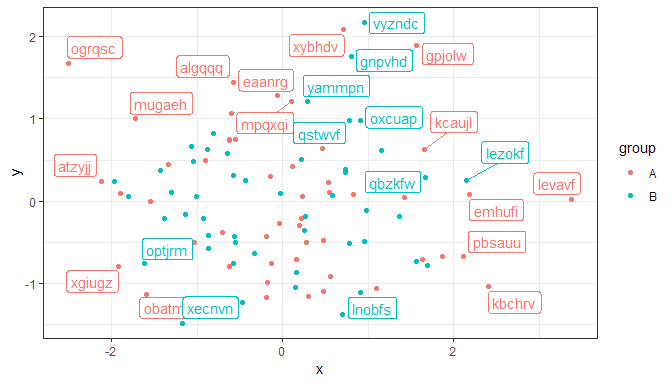Additional arguments can be used to change the angle and position of the text, but may give unexpected output when labels are long as the repulsion algorithm “sees” always a rectangular bounding box that is not rotated. With short labels or angles that are multiples of 90 degrees, there is no such problem.

ggplot(data = d, aes(x, y, label = lab, color = group)) +
geom_point() +
stat_dens2d_labels(geom = "text_repel",
keep.fraction = 0.25, angle = 90)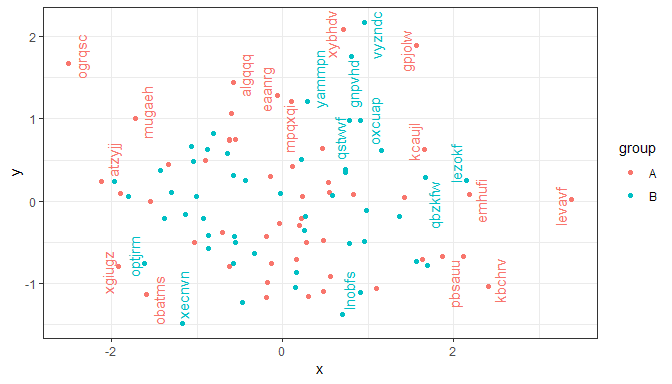Using NA as fill makes the observations with labels set to NA to be skipped completely, possibly leading to text overlapping the points corresponding to unlabelled observations around the boundary of the regions where labels are kept or discarded. We use here alpha so that the overlaps can be seen.

ggplot(data = d, aes(x, y, label = lab, color = group)) +
geom_point() +
stat_dens2d_labels(geom = "label_repel",
keep.fraction = 0.35,
alpha = 0.5,
label.fill = NA)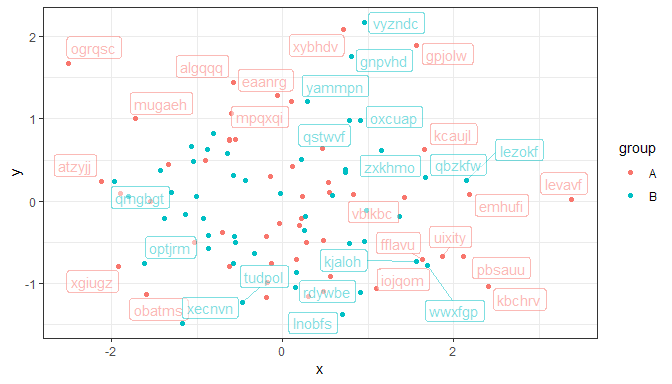try_tibble

Time series

Several different formats for storing time series data are used in R. Here we use in the examples objects of class ts but several other classes are supported as try.xts() is used internally. The first example is a quarterly series.

class(austres)
##  "ts"
austres.df <- try_tibble(austres)
class(austres.df)
##  "tbl_df"     "tbl"        "data.frame"
lapply(austres.df, "class")
## $time ##  "POSIXct" "POSIXt" ## ##$x
##  "numeric"
head(austres.df, 4)
## # A tibble: 4 x 2
##   time                     x
##   <dttm>               <dbl>
## 1 1971-04-01 00:00:00 13067.
## 2 1971-07-01 00:00:00 13130.
## 3 1971-10-01 00:00:00 13198.
## 4 1972-01-01 00:00:00 13254.

The next chunk demonstrates that numeric times are expressed as decimal years in the returned data frame.

austres.df <- try_tibble(austres, as.numeric = TRUE)
lapply(austres.df, "class")
## $time ##  "numeric" ## ##$x
##  "numeric"
head(austres.df, 4)
## # A tibble: 4 x 2
##    time      x
##   <dbl>  <dbl>
## 1 1971. 13067.
## 2 1972. 13130.
## 3 1972. 13198.
## 4 1972  13254.

This second example is for a series of yearly values.

class(lynx)
##  "ts"
lynx.df <- try_tibble(lynx)
class(lynx.df)
##  "tbl_df"     "tbl"        "data.frame"
lapply(lynx.df, "class")
## $time ##  "POSIXct" "POSIXt" ## ##$x
##  "numeric"
head(lynx.df, 3)
## # A tibble: 3 x 2
##   time                    x
##   <dttm>              <dbl>
## 1 1820-02-01 00:00:00   269
## 2 1821-02-01 00:00:00   321
## 3 1822-02-01 00:00:00   585

Above there is a small rounding error of 1 s for these old dates. We can correct this by rounding to year.

lynx.df <- try_tibble(lynx, "year")
head(lynx.df, 3)
## # A tibble: 3 x 2
##   time                    x
##   <dttm>              <dbl>
## 1 1821-01-01 00:00:00   269
## 2 1822-01-01 00:00:00   321
## 3 1823-01-01 00:00:00   585

In addition we can convert the POSIXct values into numeric values in calendar years plus a decimal fraction.

lynx_n.df <- try_tibble(lynx, "year", as.numeric = TRUE)
lapply(lynx_n.df, "class")
## $time ##  "numeric" ## ##$x
##  "numeric"
head(lynx_n.df, 3)
## # A tibble: 3 x 2
##    time     x
##   <dbl> <dbl>
## 1  1821   269
## 2  1822   321
## 3  1823   585

Other classes

try_tibble() attempts to handle gracefully objects that are not time series.

try_tibble(1:5)
## # A tibble: 5 x 1
##       x
##   <int>
## 1     1
## 2     2
## 3     3
## 4     4
## 5     5
try_tibble(letters[1:5])
## # A tibble: 5 x 1
##   x
##   <fct>
## 1 a
## 2 b
## 3 c
## 4 d
## 5 e
try_tibble(factor(letters[1:5]))
## # A tibble: 5 x 1
##   x
##   <fct>
## 1 a
## 2 b
## 3 c
## 4 d
## 5 e
try_tibble(list(x = rep(1,5), y = 1:5))
## # A tibble: 5 x 2
##       x     y
##   <dbl> <int>
## 1     1     1
## 2     1     2
## 3     1     3
## 4     1     4
## 5     1     5
try_tibble(data.frame(x = rep(1,5), y = 1:5))
## # A tibble: 5 x 2
##       x     y
##   <dbl> <int>
## 1     1     1
## 2     1     2
## 3     1     3
## 4     1     4
## 5     1     5
try_tibble(matrix(1:10, ncol = 2))
## # A tibble: 5 x 2
##      V1    V2
##   <int> <int>
## 1     1     6
## 2     2     7
## 3     3     8
## 4     4     9
## 5     5    10

Appendix: Additional examples of the use density filtering

We define a function to simplify the generation of random data sets.

make_data_tbl <- function(nrow = 100, rfun = rnorm, ...) {
if (nrow %% 2) {
nrow <- nrow + 1
}

set.seed(1001)

tibble::tibble(
x = rfun(nrow, ...),
y = rfun(nrow, ...),
group = rep(c("A", "B"), c(nrow / 2, nrow / 2))
)
}

As we will draw many points on the plotting area we change the default theme to an uncluttered one.

old_theme <- theme_set(theme_bw())

In all the examples in this vignette we use colours to demonstrate which data points are selected, but any other suitable aesthetic and discrete scale can be used instead. With keep.sparse = FALSE we keep 1/3 of the observations in the denser region of the plot. Although here we first plot all data points and later overplot the selected ones this is not necessary.

ggplot(data = make_data_tbl(300), aes(x, y)) +
geom_point() +
stat_dens2d_filter(color = "red",
keep.sparse = FALSE,
keep.fraction = 1/3)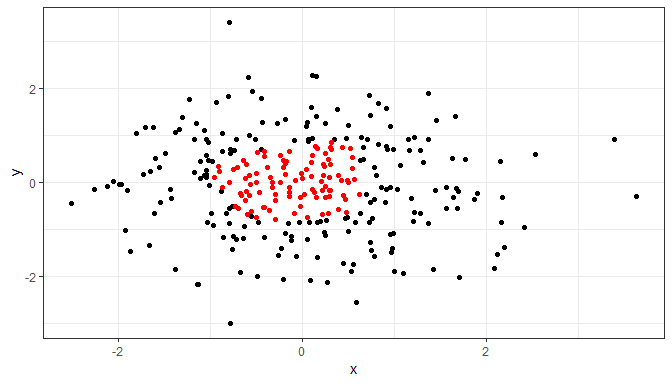Here we highlight the observations split in three group equal groups, each of a different density of observations.

ggplot(data = make_data_tbl(300), aes(x, y)) +
geom_point() +
stat_dens2d_filter(color = "red",
keep.sparse = FALSE,
keep.fraction = 1/3)+
stat_dens2d_filter(color = "blue",
keep.fraction = 1/3)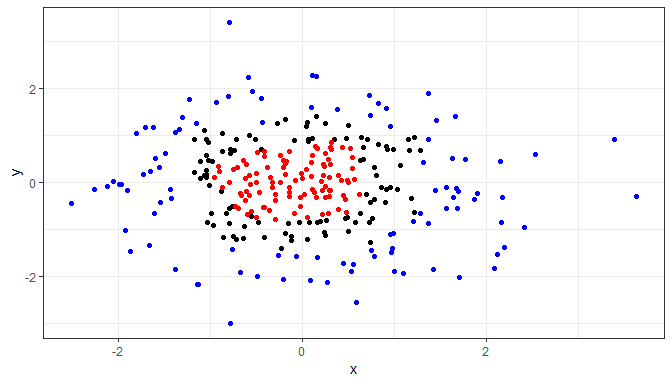The algorithm seems to work well also with other distributions, in this example the uniform distribution.

ggplot(data = make_data_tbl(300, rfun = runif), aes(x, y)) +
geom_point() +
stat_dens2d_filter(color = "red", keep.fraction = 1/2)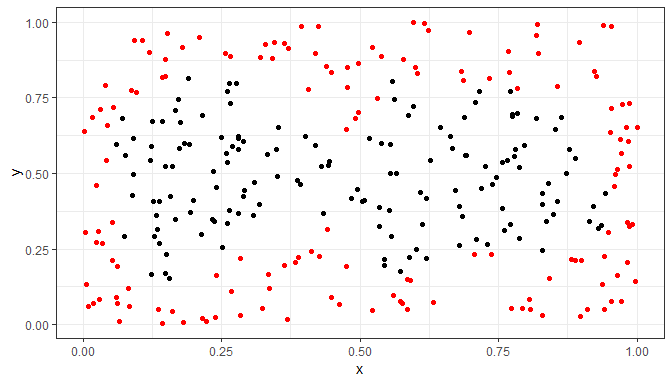One example with the gamma distribution, which is asymmetric.

ggplot(data = make_data_tbl(300, rfun = rgamma, shape = 2),
aes(x, y)) +
geom_point() +
stat_dens2d_filter(color = "red", keep.fraction = 1/3)`